Successfully reported this slideshow.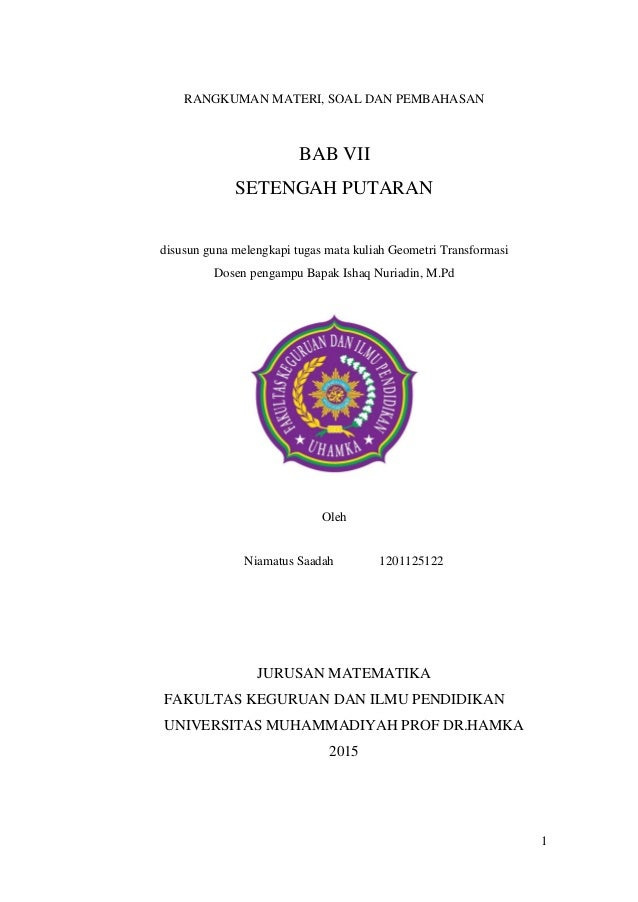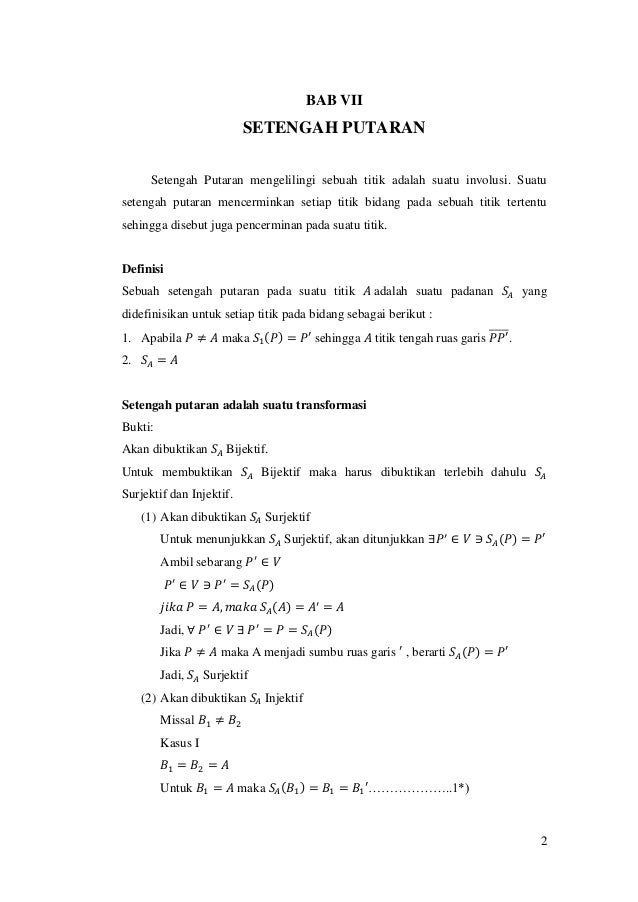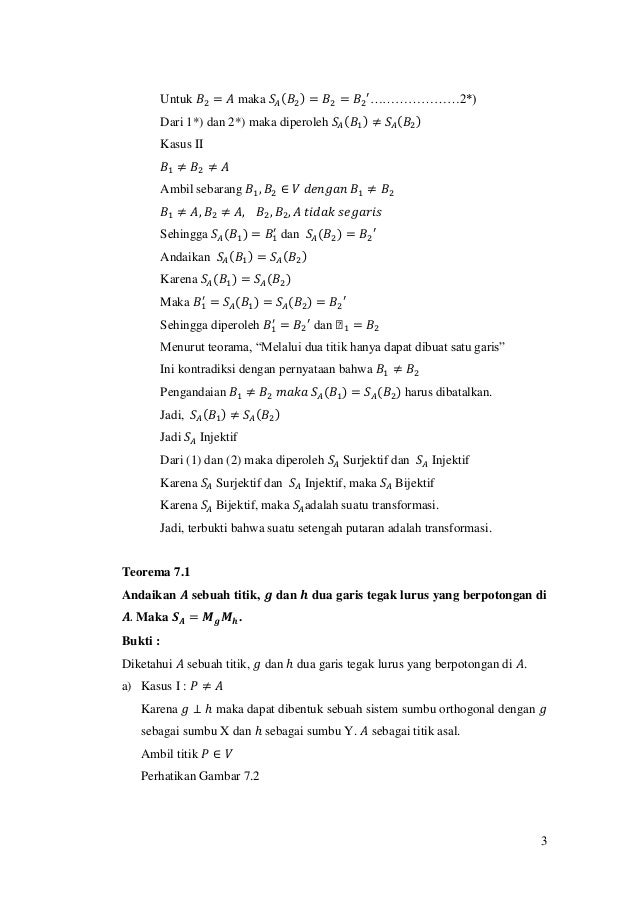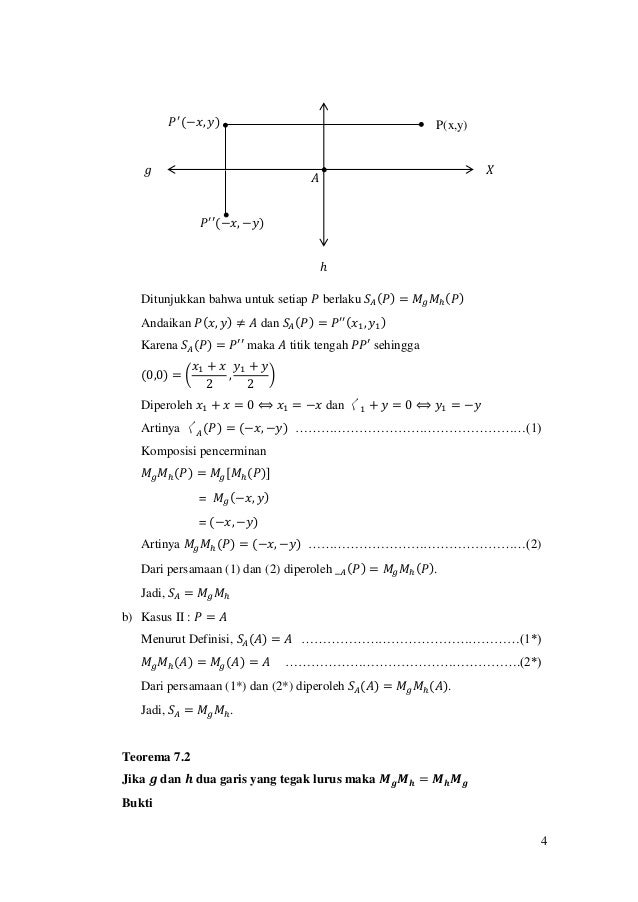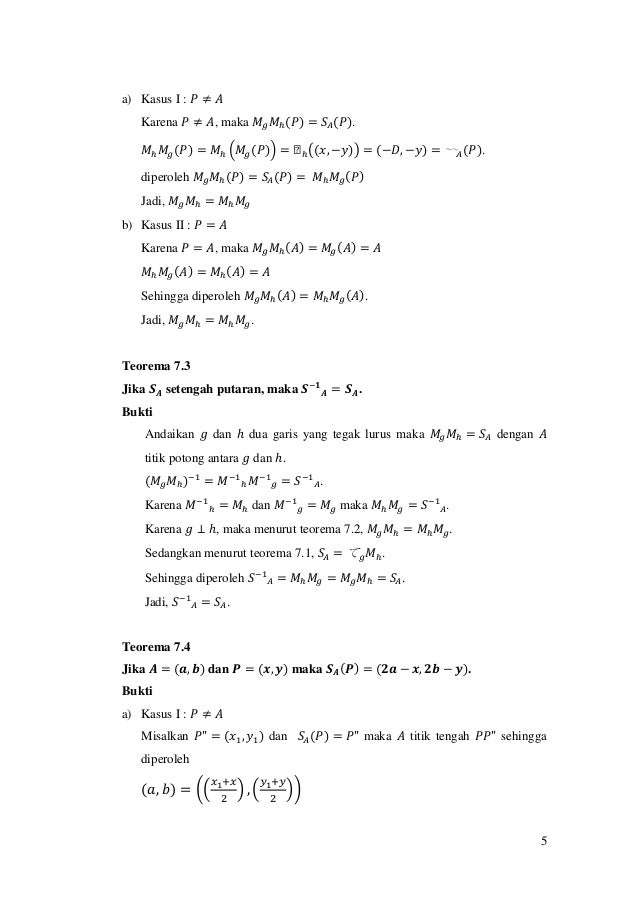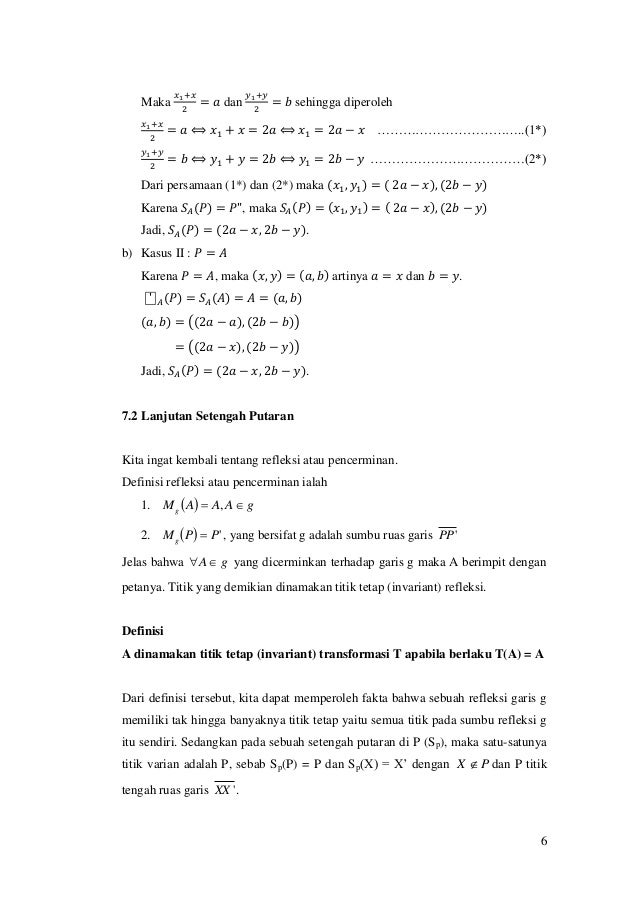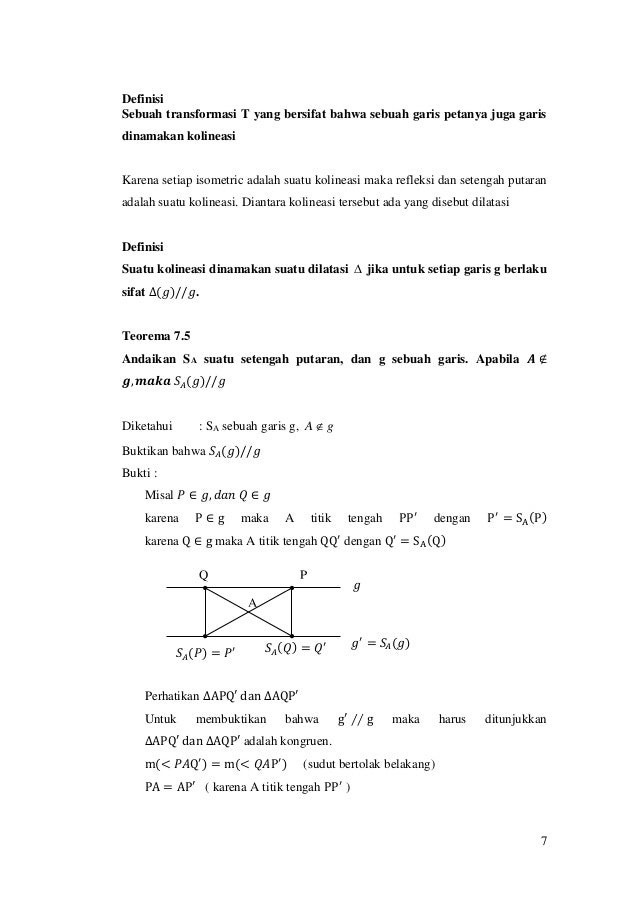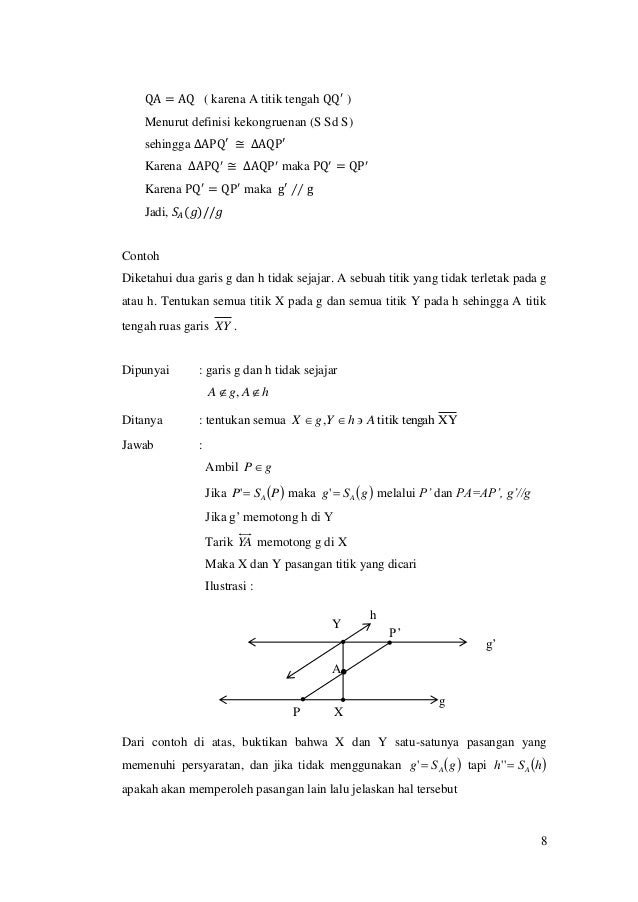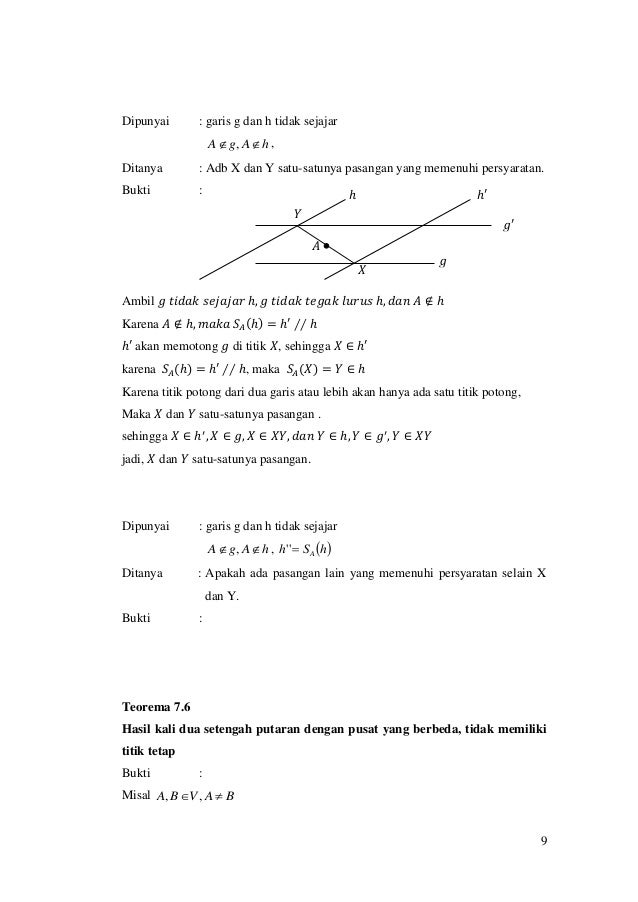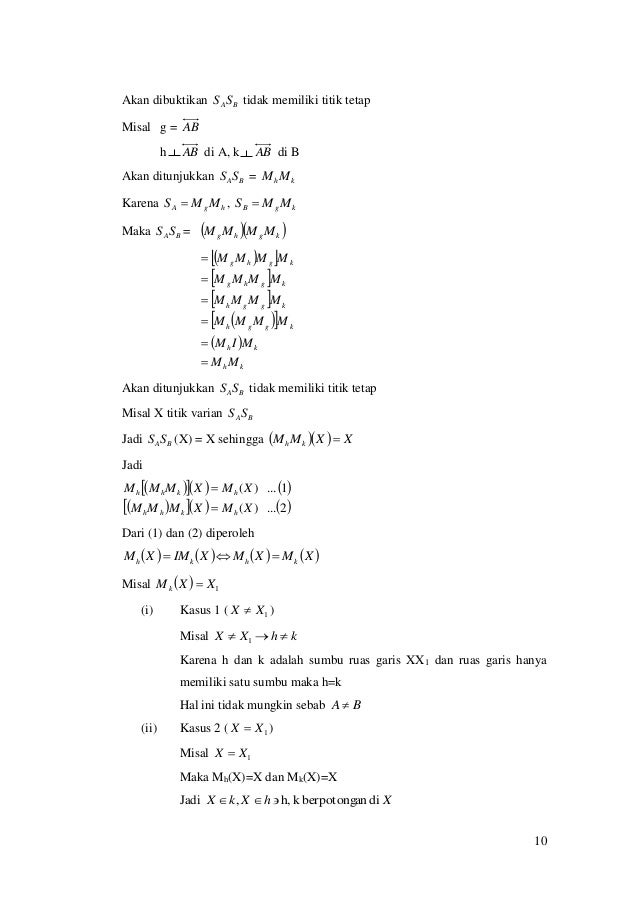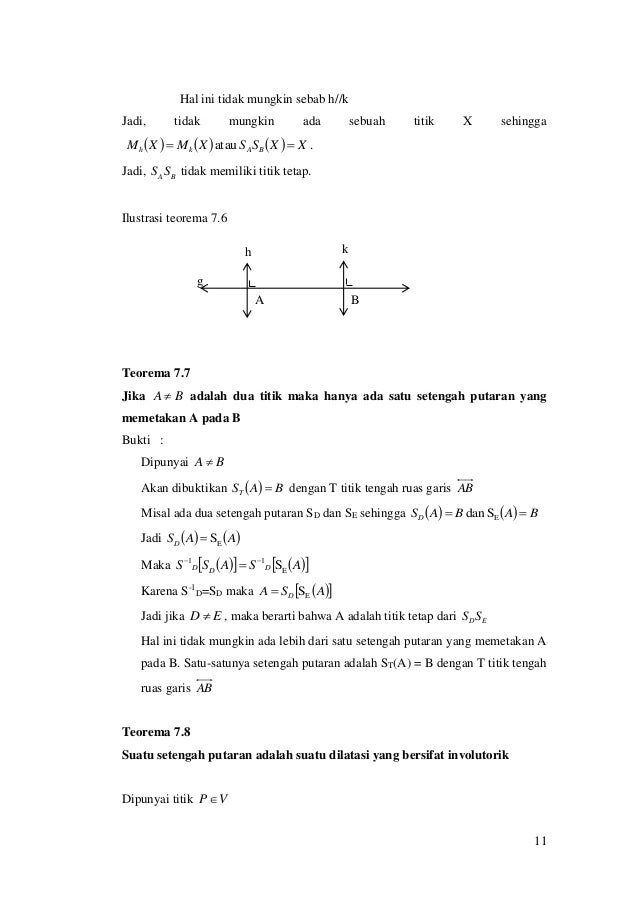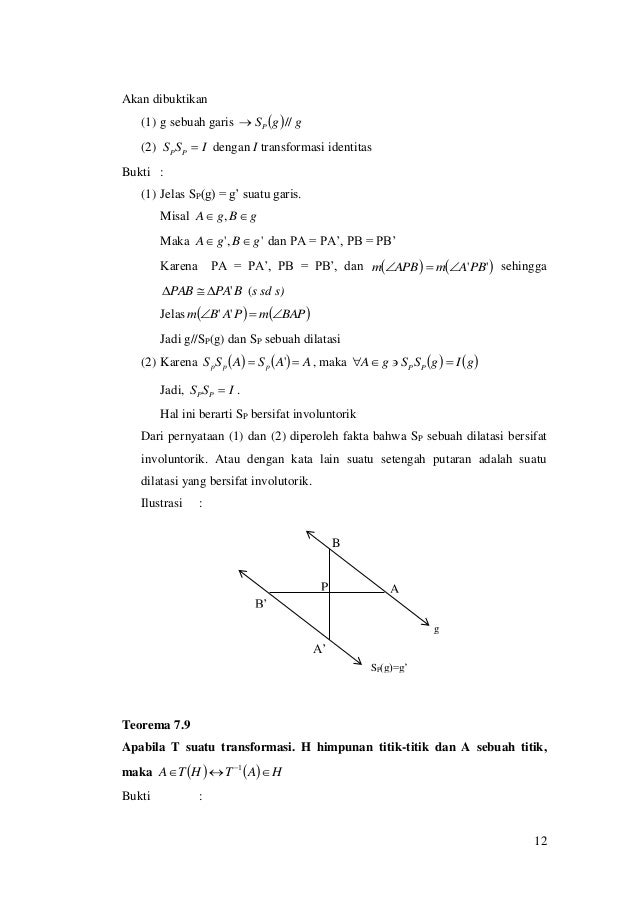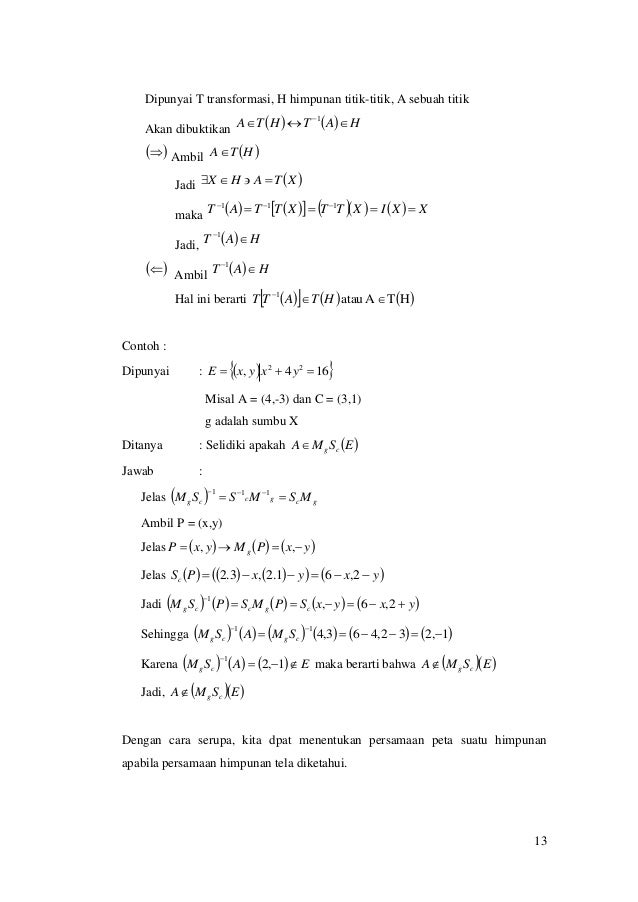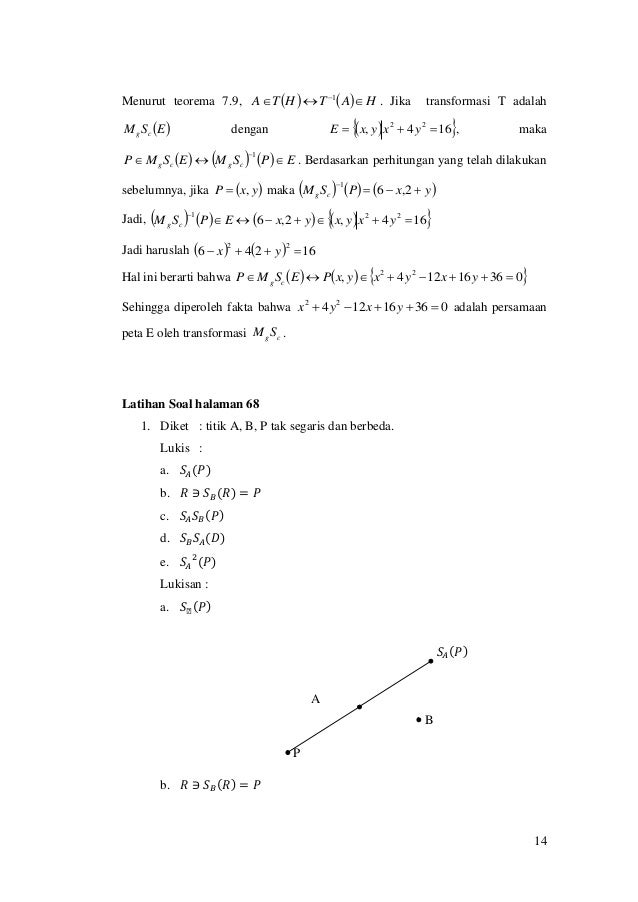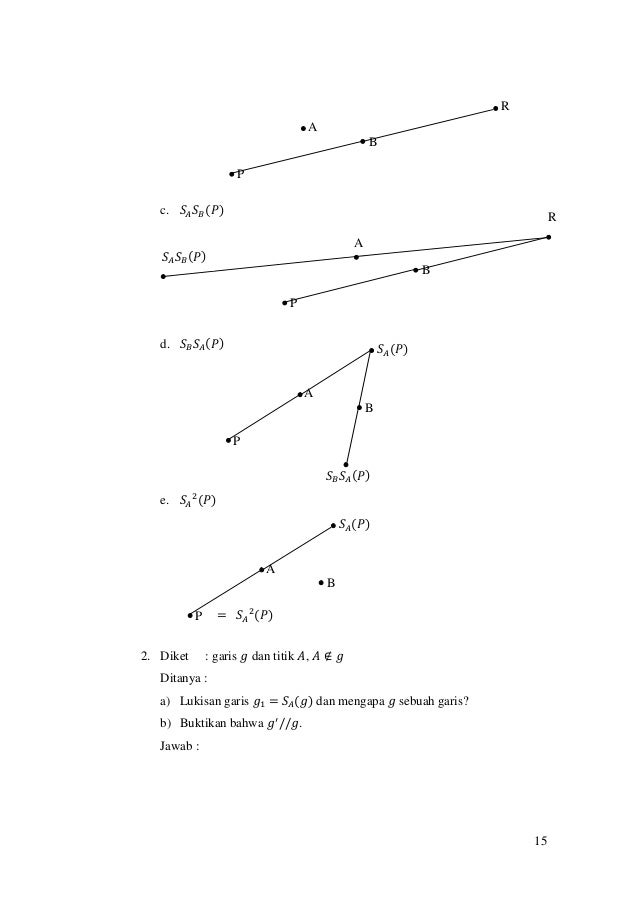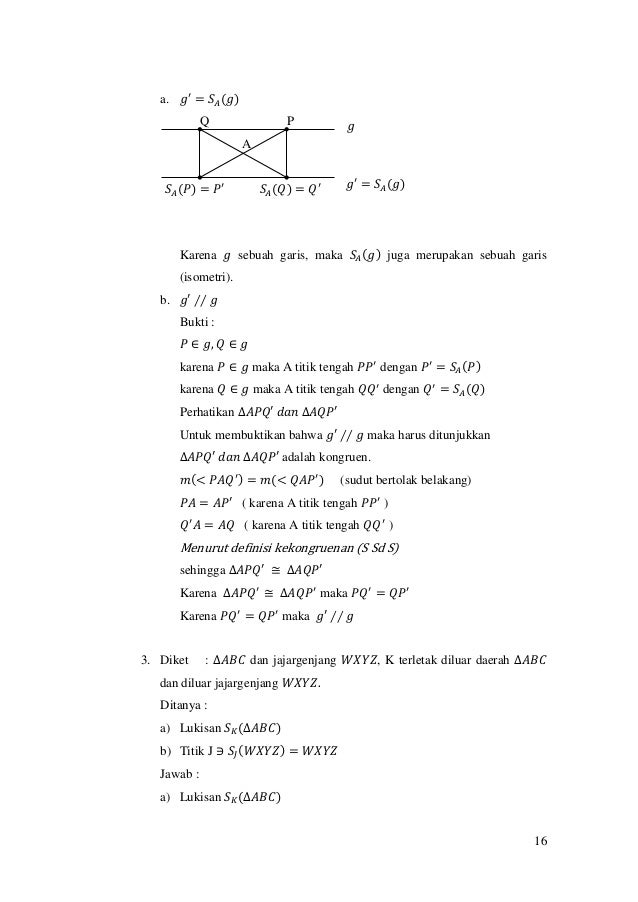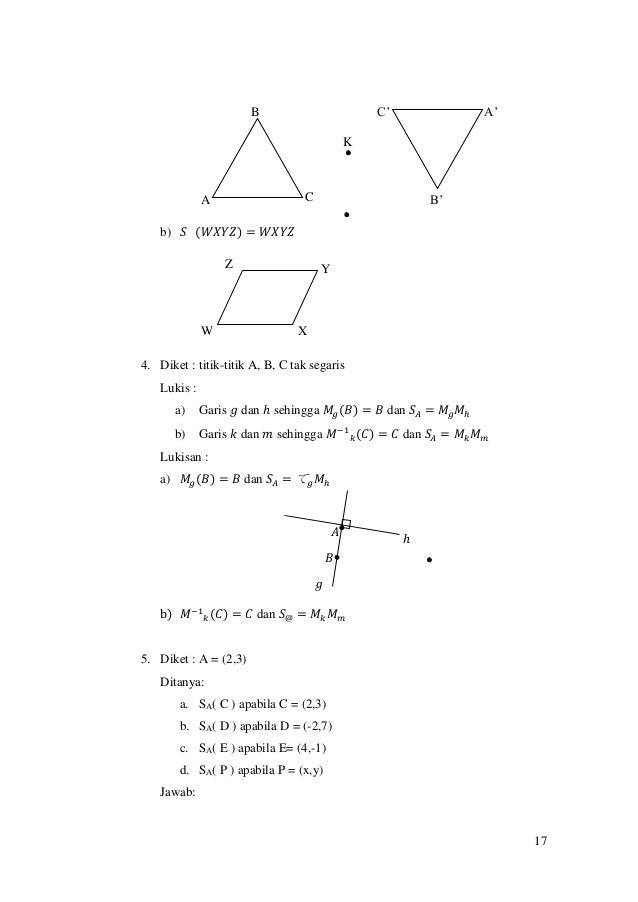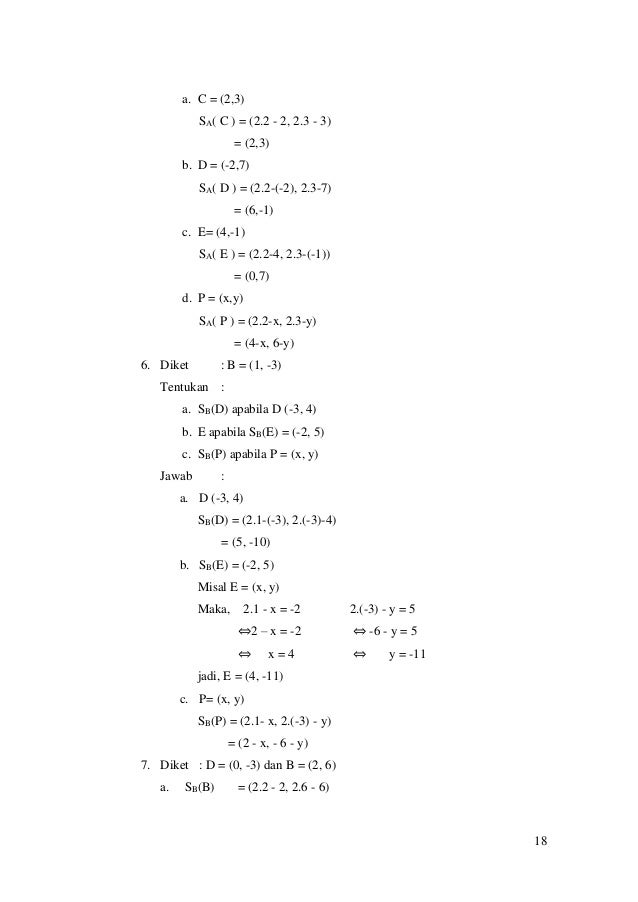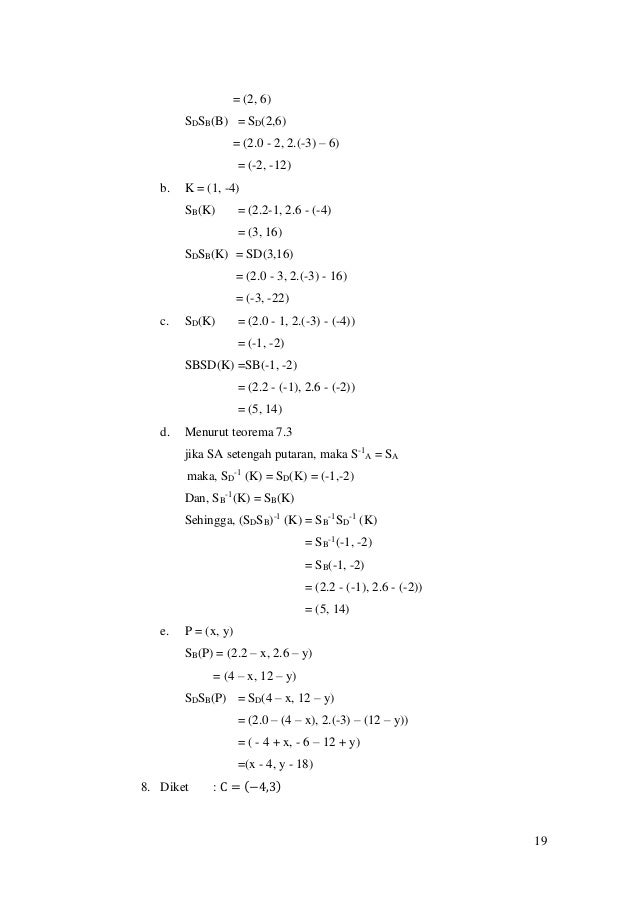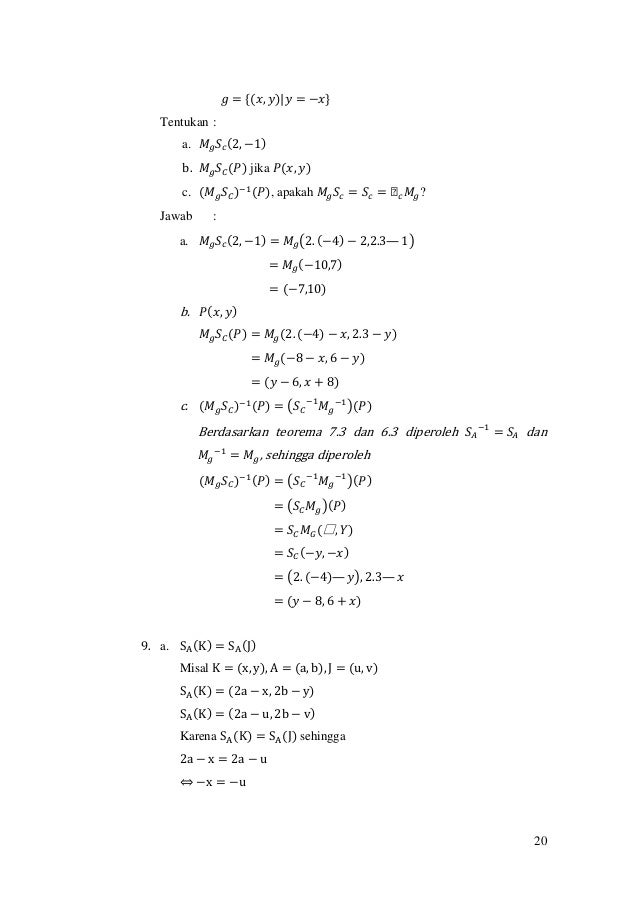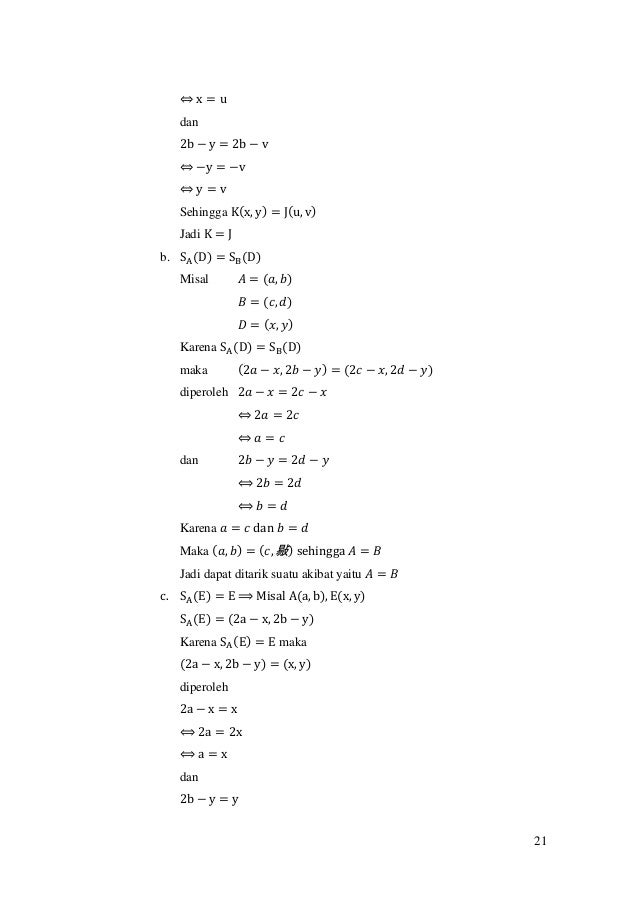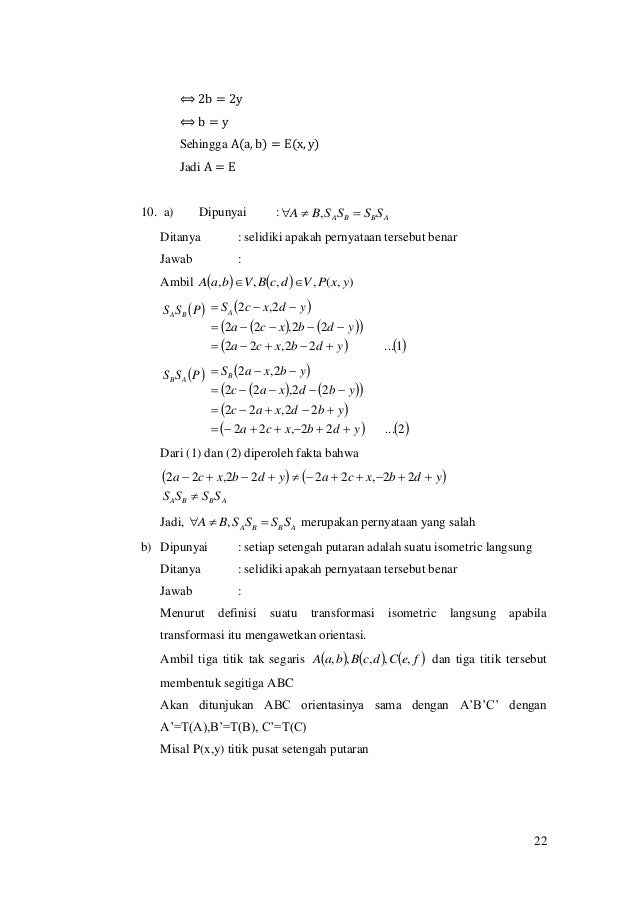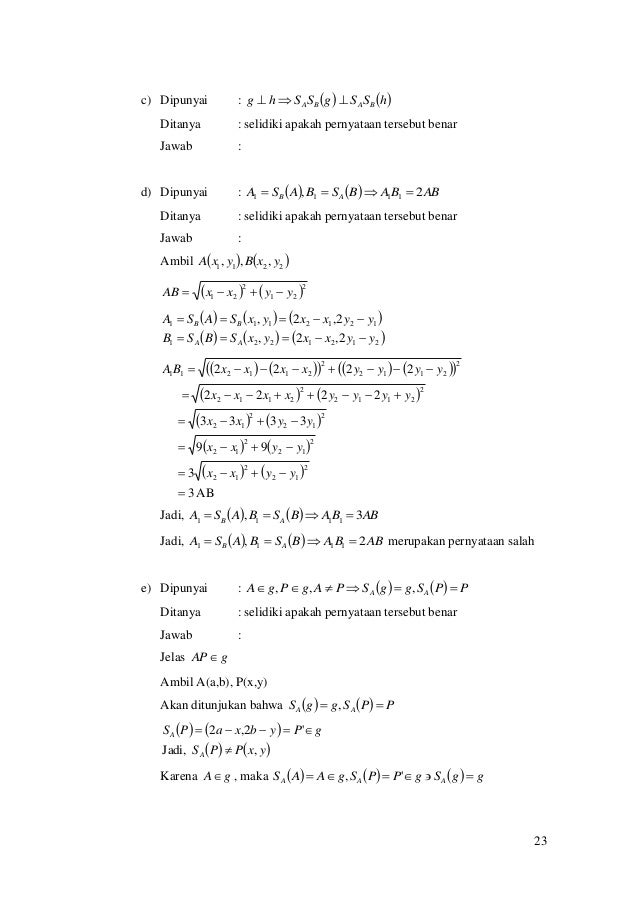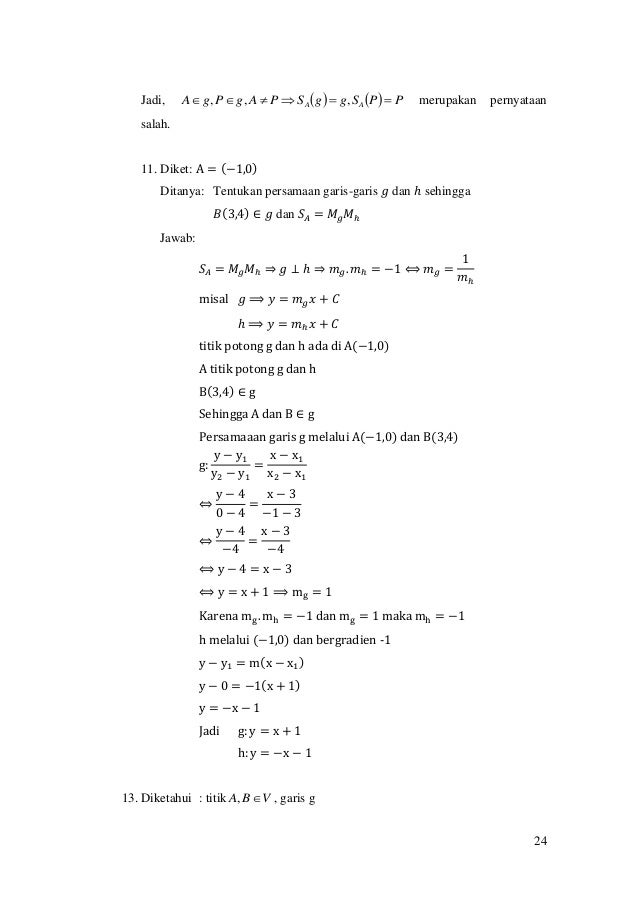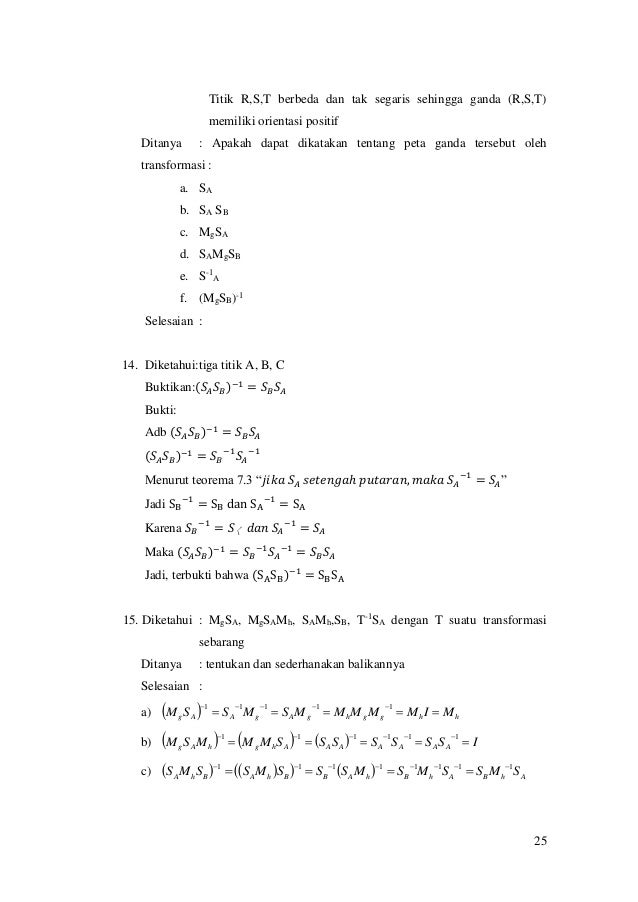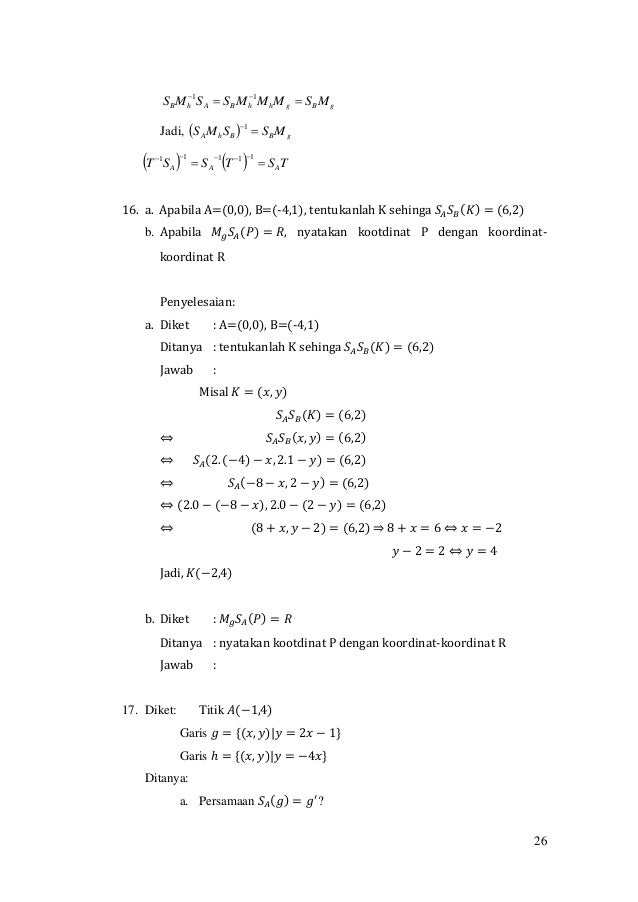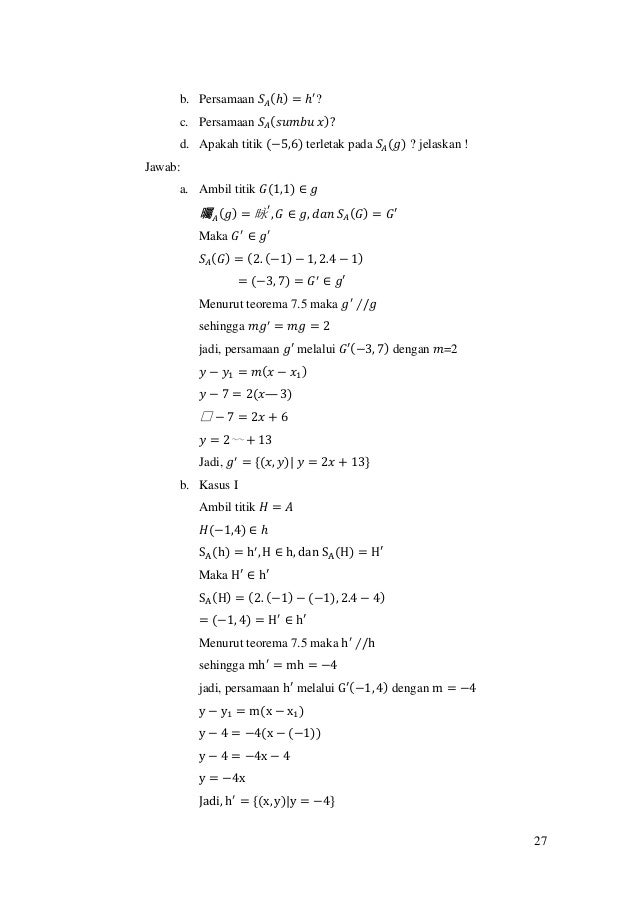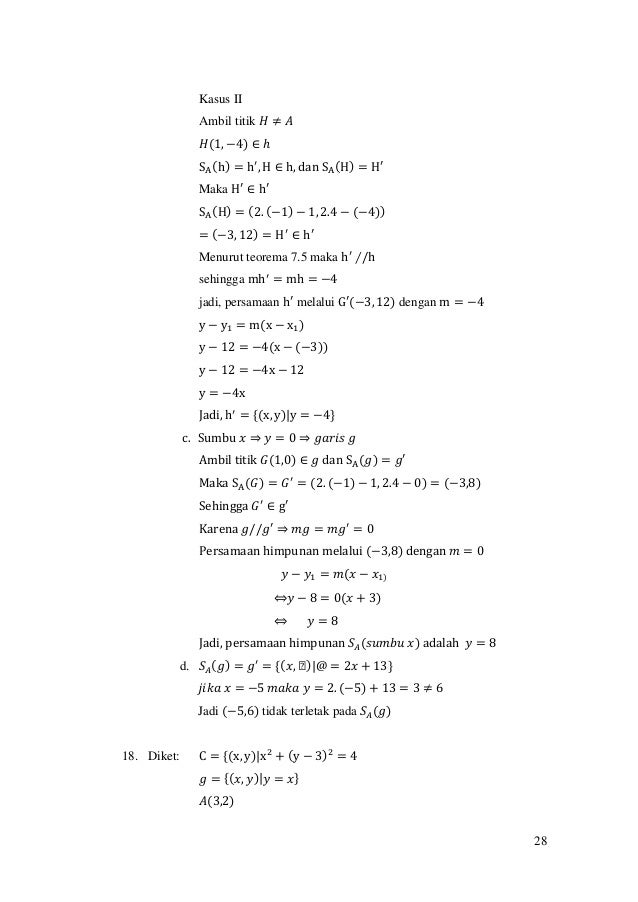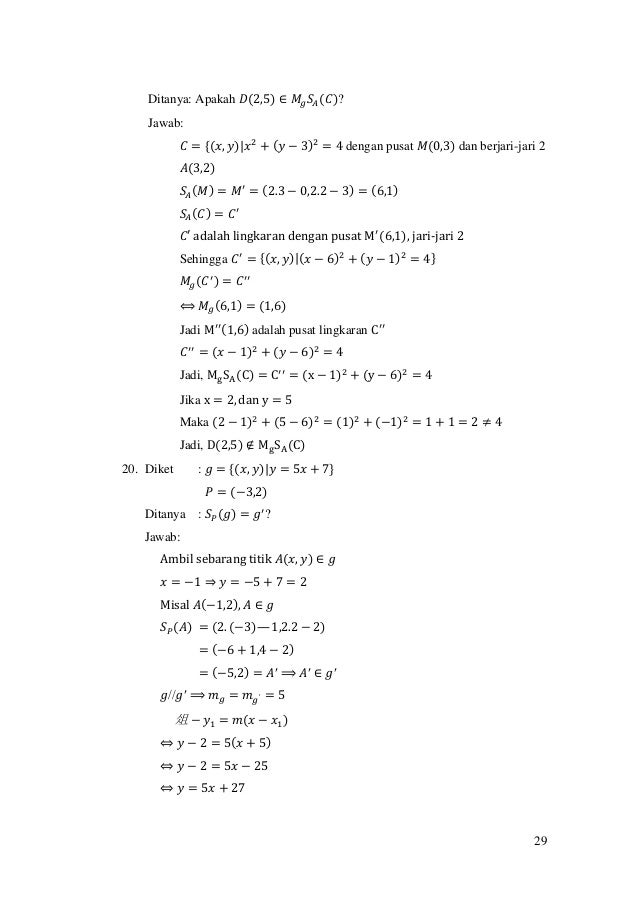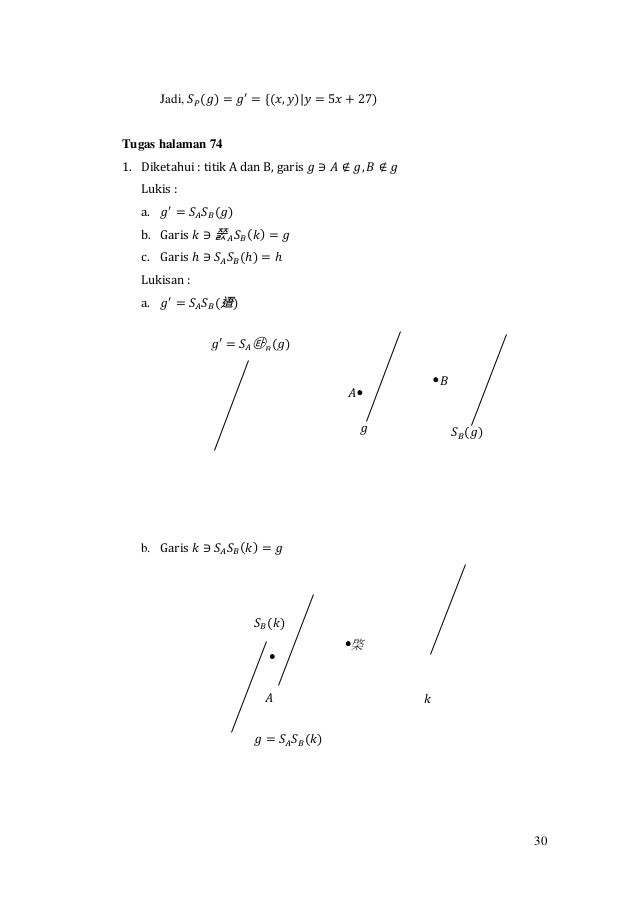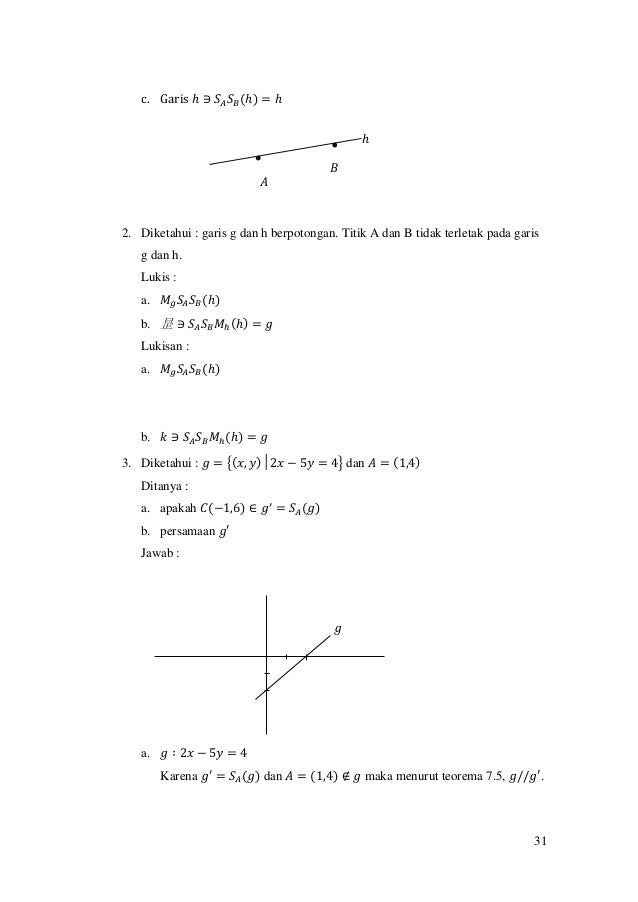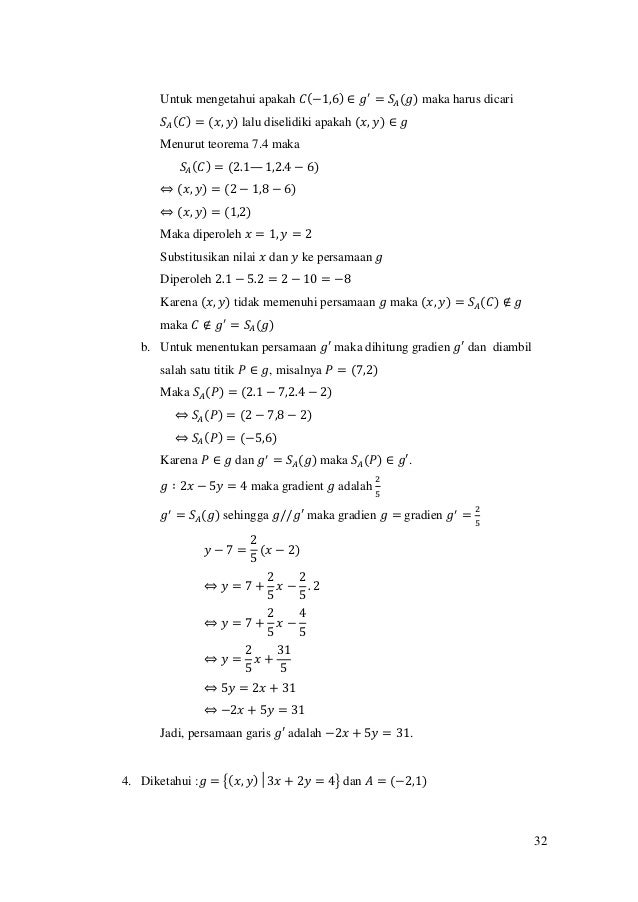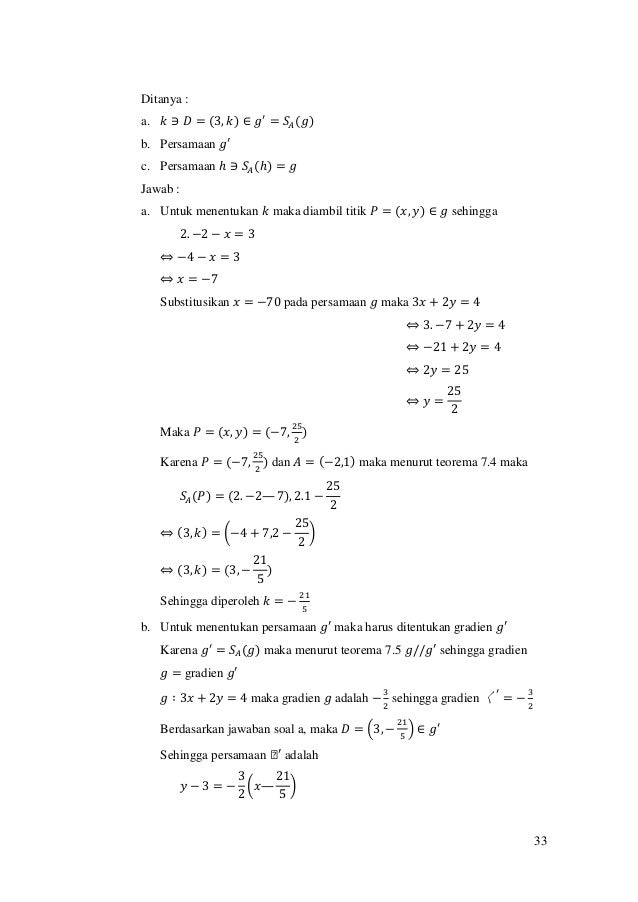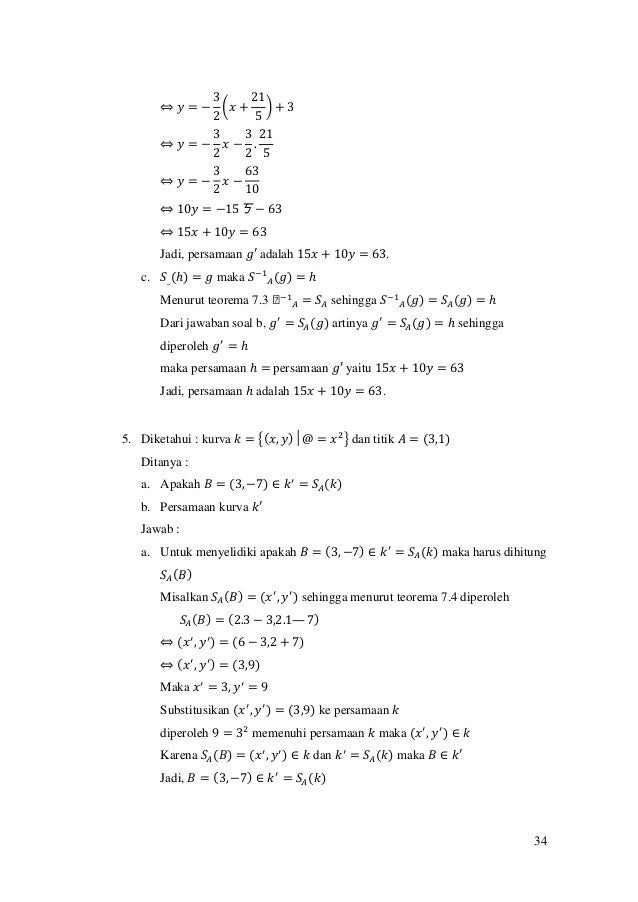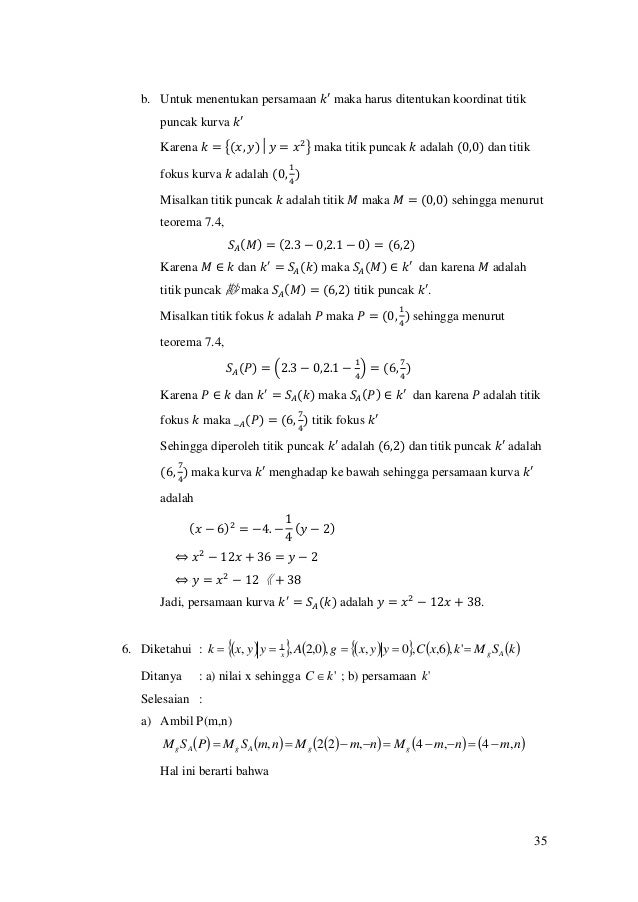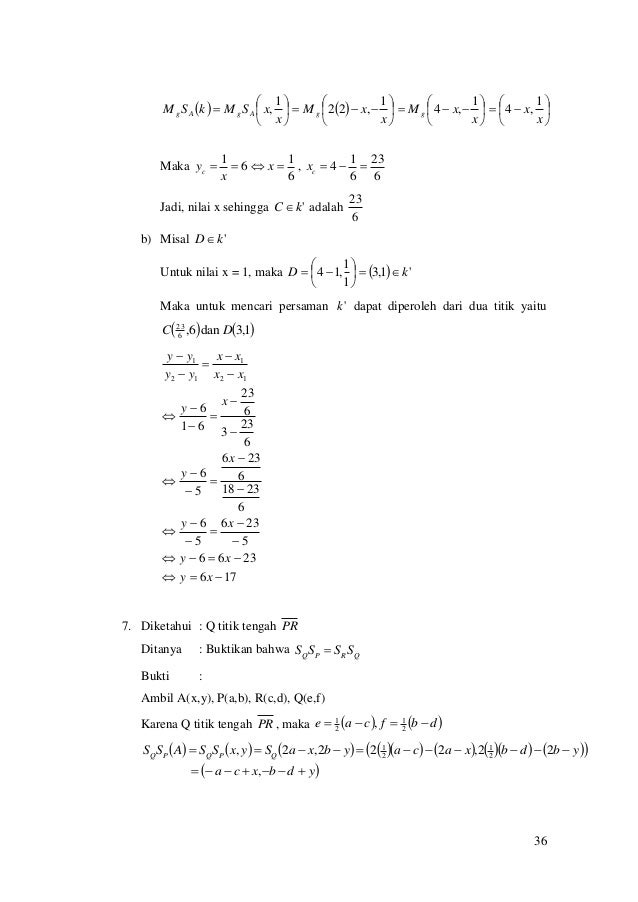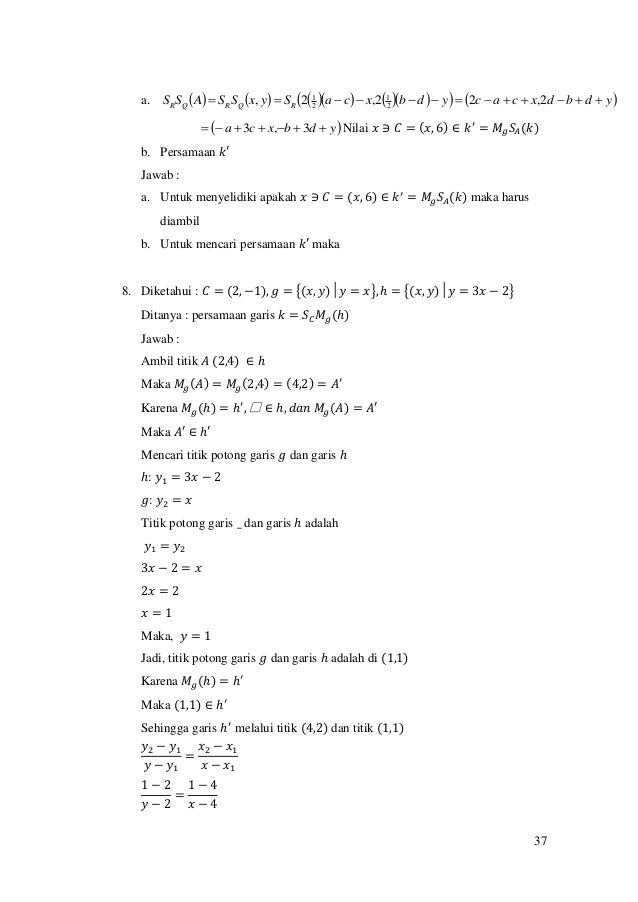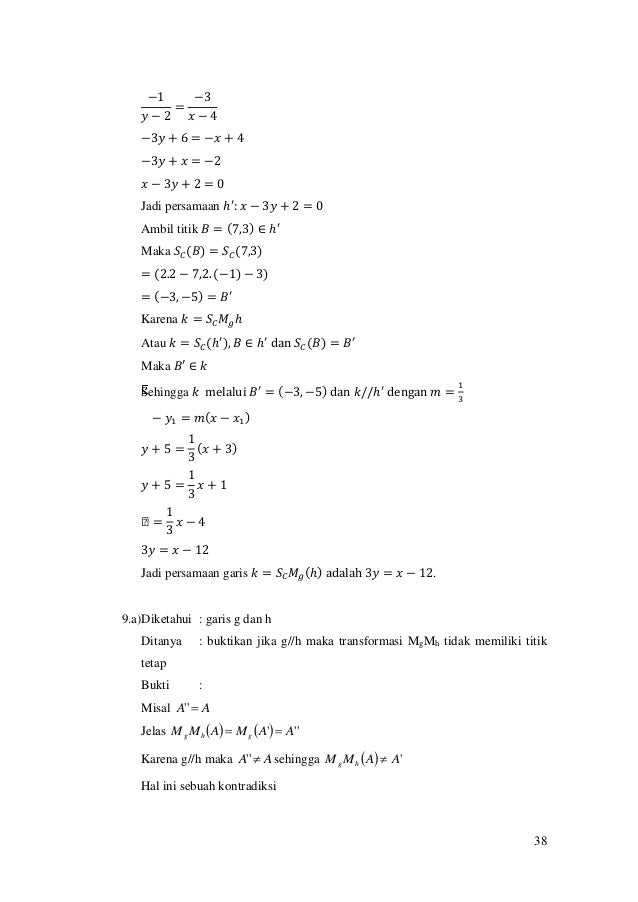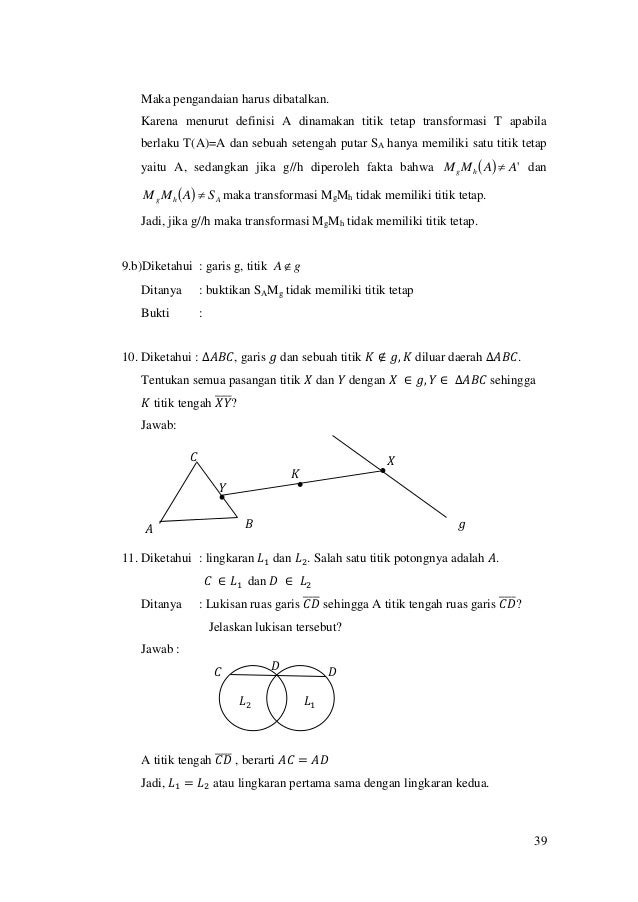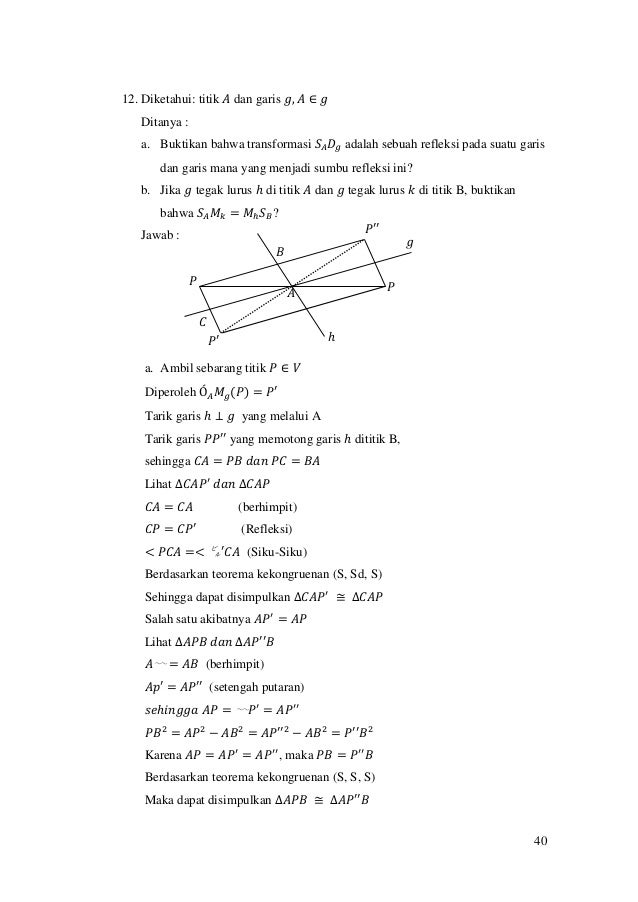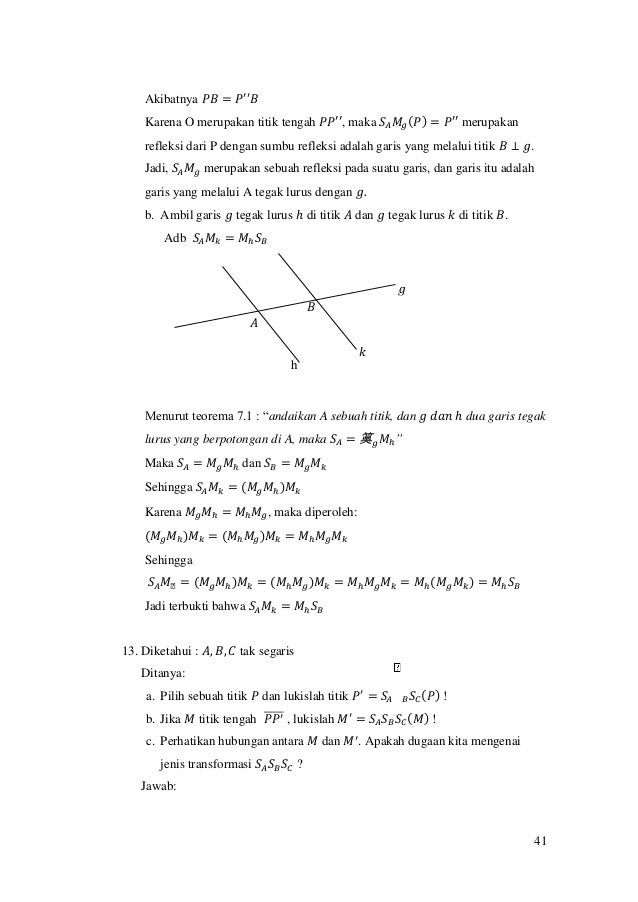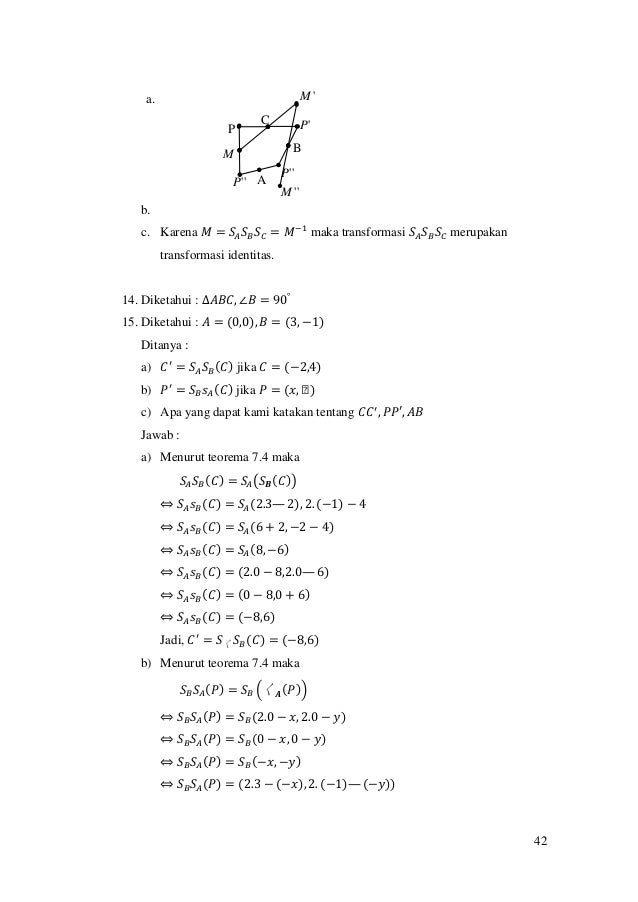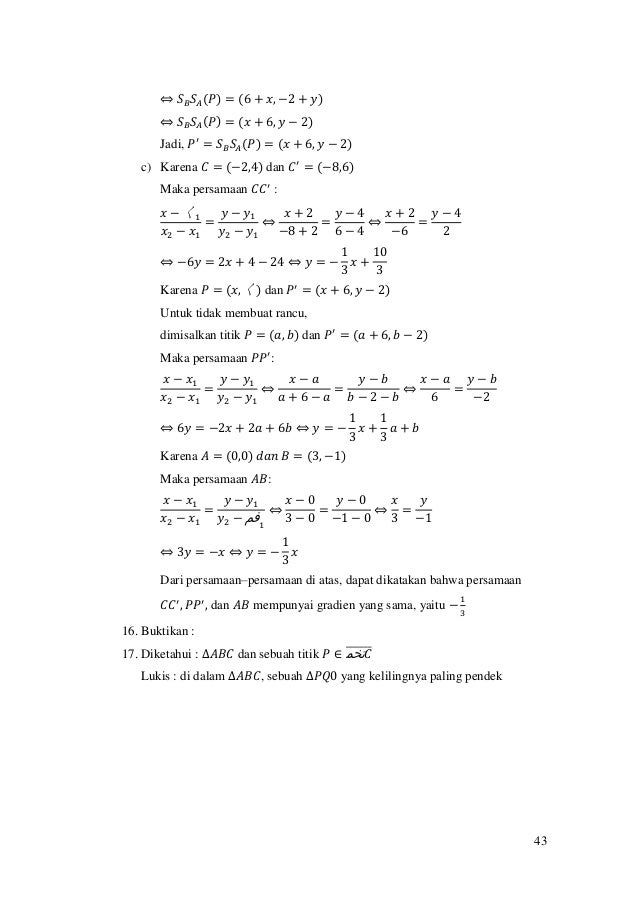Upcoming SlideShare
×

# Makalah setengah putaran

Rangkuman materi setengah putaran

• Full Name
Comment goes here.

Are you sure you want to Yes No• Be the first to comment

### Makalah setengah putaran

1. 1. 1 RANGKUMAN MATERI, SOAL DAN PEMBAHASAN BAB VII SETENGAH PUTARAN disusun guna melengkapi tugas mata kuliah Geometri Transformasi Dosen pengampu Bapak Ishaq Nuriadin, M.Pd Oleh Niamatus Saadah 1201125122 JURUSAN MATEMATIKA FAKULTAS KEGURUAN DAN ILMU PENDIDIKAN UNIVERSITAS MUHAMMADIYAH PROF DR.HAMKA 2015
2. 2. 2 BAB VII SETENGAH PUTARAN Setengah Putaran mengelilingi sebuah titik adalah suatu involusi. Suatu setengah putaran mencerminkan setiap titik bidang pada sebuah titik tertentu sehingga disebut juga pencerminan pada suatu titik. Definisi Sebuah setengah putaran pada suatu titik 𝐴 adalah suatu padanan 𝑆𝐴 yang didefinisikan untuk setiap titik pada bidang sebagai berikut : 1. Apabila 𝑃 ≠ 𝐴 maka 𝑆1(𝑃) = 𝑃′ sehingga 𝐴 titik tengah ruas garis 𝑃𝑃′̅̅̅̅̅. 2. 𝑆𝐴 = 𝐴 Setengah putaran adalah suatu transformasi Bukti: Akan dibuktikan 𝑆𝐴 Bijektif. Untuk membuktikan 𝑆𝐴 Bijektif maka harus dibuktikan terlebih dahulu 𝑆𝐴 Surjektif dan Injektif. (1) Akan dibuktikan 𝑆𝐴 Surjektif Untuk menunjukkan 𝑆𝐴 Surjektif, akan ditunjukkan ∃𝑃′ ∈ 𝑉 ∋ 𝑆𝐴(𝑃) = 𝑃′ Ambil sebarang 𝑃′ ∈ 𝑉 𝑃′ ∈ 𝑉 ∋ 𝑃′ = 𝑆𝐴(𝑃) 𝑗𝑖𝑘𝑎 𝑃 = 𝐴, 𝑚𝑎𝑘𝑎 𝑆𝐴(𝐴) = 𝐴′ = 𝐴 Jadi, ∀ 𝑃′ ∈ 𝑉 ∃ 𝑃′ = 𝑃 = 𝑆𝐴(𝑃) Jika 𝑃 ≠ 𝐴 maka A menjadi sumbu ruas garis ′ , berarti 𝑆𝐴(𝑃) = 𝑃′ Jadi, 𝑆𝐴 Surjektif (2) Akan dibuktikan 𝑆𝐴 Injektif Missal 𝐵1 ≠ 𝐵2 Kasus I 𝐵1 = 𝐵2 = 𝐴 Untuk 𝐵1 = 𝐴 maka 𝑆𝐴(𝐵1) = 𝐵1 = 𝐵1′………………..1*)
3. 3. 3 Untuk 𝐵2 = 𝐴 maka 𝑆𝐴(𝐵2) = 𝐵2 = 𝐵2′…………………2*) Dari 1*) dan 2*) maka diperoleh 𝑆𝐴(𝐵1) ≠ 𝑆𝐴(𝐵2) Kasus II 𝐵1 ≠ 𝐵2 ≠ 𝐴 Ambil sebarang 𝐵1, 𝐵2 ∈ 𝑉 𝑑𝑒𝑛𝑔𝑎𝑛 𝐵1 ≠ 𝐵2 𝐵1 ≠ 𝐴, 𝐵2 ≠ 𝐴, 𝐵2, 𝐵2, 𝐴 𝑡𝑖𝑑𝑎𝑘 𝑠𝑒𝑔𝑎𝑟𝑖𝑠 Sehingga 𝑆𝐴(𝐵1) = 𝐵1 ′ dan 𝑆𝐴(𝐵2) = 𝐵2′ Andaikan 𝑆𝐴(𝐵1) = 𝑆 𝐴(𝐵2) Karena 𝑆𝐴(𝐵1) = 𝑆 𝐴(𝐵2) Maka 𝐵1 ′ = 𝑆𝐴(𝐵1) = 𝑆𝐴(𝐵2) = 𝐵2′ Sehingga diperoleh 𝐵1 ′ = 𝐵2′ dan ᒐ1 = 𝐵2 Menurut teorama, “Melalui dua titik hanya dapat dibuat satu garis” Ini kontradiksi dengan pernyataan bahwa 𝐵1 ≠ 𝐵2 Pengandaian 𝐵1 ≠ 𝐵2 𝑚𝑎𝑘𝑎 𝑆𝐴(𝐵1) = 𝑆 𝐴(𝐵2) harus dibatalkan. Jadi, 𝑆𝐴(𝐵1) ≠ 𝑆𝐴(𝐵2) Jadi 𝑆𝐴 Injektif Dari (1) dan (2) maka diperoleh 𝑆𝐴 Surjektif dan 𝑆𝐴 Injektif Karena 𝑆𝐴 Surjektif dan 𝑆𝐴 Injektif, maka 𝑆𝐴 Bijektif Karena 𝑆𝐴 Bijektif, maka 𝑆𝐴adalah suatu transformasi. Jadi, terbukti bahwa suatu setengah putaran adalah transformasi. Teorema 7.1 Andaikan 𝑨 sebuah titik, 𝒈 dan 𝒉 dua garis tegak lurus yang berpotongan di 𝑨. Maka 𝑺 𝑨 = 𝑴 𝒈 𝑴 𝒉. Bukti : Diketahui 𝐴 sebuah titik, 𝑔 dan ℎ dua garis tegak lurus yang berpotongan di 𝐴. a) Kasus I : 𝑃 ≠ 𝐴 Karena 𝑔 ⊥ ℎ maka dapat dibentuk sebuah sistem sumbu orthogonal dengan 𝑔 sebagai sumbu X dan ℎ sebagai sumbu Y. 𝐴 sebagai titik asal. Ambil titik 𝑃 ∈ 𝑉 Perhatikan Gambar 7.2
4. 4. 4 Ditunjukkan bahwa untuk setiap 𝑃 berlaku 𝑆𝐴(𝑃) = 𝑀𝑔 𝑀ℎ(𝑃) Andaikan 𝑃(𝑥, 𝑦) ≠ 𝐴 dan 𝑆𝐴(𝑃) = 𝑃′′(𝑥1, 𝑦1) Karena 𝑆𝐴(𝑃) = 𝑃′′ maka 𝐴 titik tengah 𝑃𝑃′ sehingga (0,0) = ( 𝑥1 + 𝑥 2 , 𝑦1 + 𝑦 2 ) Diperoleh 𝑥1 + 𝑥 = 0 ⟺ 𝑥1 = −𝑥 dan 〱1 + 𝑦 = 0 ⟺ 𝑦1 = −𝑦 Artinya 〱 𝐴(𝑃) = (−𝑥, −𝑦) ………………………………………………(1) Komposisi pencerminan 𝑀𝑔 𝑀ℎ(𝑃) = 𝑀𝑔[𝑀ℎ(𝑃)] = 𝑀𝑔(−𝑥, 𝑦) = (−𝑥, −𝑦) Artinya 𝑀𝑔 𝑀ℎ(𝑃) = (−𝑥, −𝑦) ……………………………………………(2) Dari persamaan (1) dan (2) diperoleh _ 𝐴(𝑃) = 𝑀𝑔 𝑀ℎ(𝑃). Jadi, 𝑆𝐴 = 𝑀𝑔 𝑀ℎ b) Kasus II : 𝑃 = 𝐴 Menurut Definisi, 𝑆𝐴(𝐴) = 𝐴 ……………………………………………(1*) 𝑀𝑔 𝑀ℎ(𝐴) = 𝑀𝑔(𝐴) = 𝐴 ……………………………………………….(2*) Dari persamaan (1*) dan (2*) diperoleh 𝑆𝐴(𝐴) = 𝑀𝑔 𝑀ℎ(𝐴). Jadi, 𝑆𝐴 = 𝑀𝑔 𝑀ℎ. Teorema 7.2 Jika 𝒈 dan 𝒉 dua garis yang tegak lurus maka 𝑴 𝒈 𝑴 𝒉 = 𝑴 𝒉 𝑴 𝒈 Bukti 𝐴 𝑃′ ′(−𝑥, −𝑦) 𝑃′ (−𝑥, 𝑦) P(x,y) ℎ 𝑔 𝑋
5. 5. 5 a) Kasus I : 𝑃 ≠ 𝐴 Karena 𝑃 ≠ 𝐴, maka 𝑀𝑔 𝑀ℎ(𝑃) = 𝑆𝐴(𝑃). 𝑀ℎ 𝑀𝑔(𝑃) = 𝑀ℎ (𝑀𝑔(𝑃)) = ᒐℎ((𝑥, −𝑦)) = (−𝐷, −𝑦) = 〰 𝐴(𝑃). diperoleh 𝑀𝑔 𝑀ℎ(𝑃) = 𝑆𝐴(𝑃) = 𝑀ℎ 𝑀𝑔(𝑃) Jadi, 𝑀𝑔 𝑀ℎ = 𝑀ℎ 𝑀𝑔 b) Kasus II : 𝑃 = 𝐴 Karena 𝑃 = 𝐴, maka 𝑀𝑔 𝑀ℎ(𝐴) = 𝑀𝑔(𝐴) = 𝐴 𝑀ℎ 𝑀𝑔(𝐴) = 𝑀ℎ(𝐴) = 𝐴 Sehingga diperoleh 𝑀𝑔 𝑀ℎ(𝐴) = 𝑀ℎ 𝑀𝑔(𝐴). Jadi, 𝑀𝑔 𝑀ℎ = 𝑀ℎ 𝑀𝑔. Teorema 7.3 Jika 𝑺 𝑨 setengah putaran, maka 𝑺−𝟏 𝑨 = 𝑺 𝑨. Bukti Andaikan 𝑔 dan ℎ dua garis yang tegak lurus maka 𝑀𝑔 𝑀ℎ = 𝑆𝐴 dengan 𝐴 titik potong antara 𝑔 dan ℎ. (𝑀𝑔 𝑀ℎ)−1 = 𝑀−1 ℎ 𝑀−1 𝑔 = 𝑆−1 𝐴. Karena 𝑀−1 ℎ = 𝑀ℎ dan 𝑀−1 𝑔 = 𝑀𝑔 maka 𝑀ℎ 𝑀𝑔 = 𝑆−1 𝐴. Karena 𝑔 ⊥ ℎ, maka menurut teorema 7.2, 𝑀𝑔 𝑀ℎ = 𝑀ℎ 𝑀𝑔. Sedangkan menurut teorema 7.1, 𝑆𝐴 = て 𝑔 𝑀ℎ. Sehingga diperoleh 𝑆−1 𝐴 = 𝑀ℎ 𝑀𝑔 = 𝑀𝑔 𝑀ℎ = 𝑆𝐴. Jadi, 𝑆−1 𝐴 = 𝑆𝐴. Teorema 7.4 Jika 𝑨 = (𝒂, 𝒃) dan 𝑷 = (𝒙, 𝒚) maka 𝑺 𝑨(𝑷) = (𝟐𝒂 − 𝒙, 𝟐𝒃 − 𝒚). Bukti a) Kasus I : 𝑃 ≠ 𝐴 Misalkan 𝑃" = (𝑥1, 𝑦1) dan 𝑆𝐴(𝑃) = 𝑃" maka 𝐴 titik tengah 𝑃𝑃" sehingga diperoleh (𝑎, 𝑏) = (( 𝑥1+𝑥 2 ) , ( 𝑦1+𝑦 2 ))
6. 6. 6 Maka 𝑥1+𝑥 2 = 𝑎 dan 𝑦1+𝑦 2 = 𝑏 sehingga diperoleh 𝑥1+𝑥 2 = 𝑎 ⟺ 𝑥1 + 𝑥 = 2𝑎 ⟺ 𝑥1 = 2𝑎 − 𝑥 ……………………………..(1*) 𝑦1+𝑦 2 = 𝑏 ⟺ 𝑦1 + 𝑦 = 2𝑏 ⟺ 𝑦1 = 2𝑏 − 𝑦 ………………………………(2*) Dari persamaan (1*) dan (2*) maka (𝑥1, 𝑦1) = ( 2𝑎 − 𝑥), (2𝑏 − 𝑦) Karena 𝑆𝐴(𝑃) = 𝑃", maka 𝑆𝐴(𝑃) = (𝑥1, 𝑦1) = ( 2𝑎 − 𝑥), (2𝑏 − 𝑦) Jadi, 𝑆𝐴(𝑃) = (2𝑎 − 𝑥, 2𝑏 − 𝑦). b) Kasus II : 𝑃 = 𝐴 Karena 𝑃 = 𝐴, maka (𝑥, 𝑦) = (𝑎, 𝑏) artinya 𝑎 = 𝑥 dan 𝑏 = 𝑦. ⍞ 𝐴(𝑃) = 𝑆𝐴(𝐴) = 𝐴 = (𝑎, 𝑏) (𝑎, 𝑏) = ((2𝑎 − 𝑎), (2𝑏 − 𝑏)) = ((2𝑎 − 𝑥), (2𝑏 − 𝑦)) Jadi, 𝑆𝐴(𝑃) = (2𝑎 − 𝑥, 2𝑏 − 𝑦). 7.2 Lanjutan Setengah Putaran Kita ingat kembali tentang refleksi atau pencerminan. Definisi refleksi atau pencerminan ialah 1.   gAAAMg  , 2.   'PPMg  , yang bersifat g adalah sumbu ruas garis 'PP Jelas bahwa gA yang dicerminkan terhadap garis g maka A berimpit dengan petanya. Titik yang demikian dinamakan titik tetap (invariant) refleksi. Definisi A dinamakan titik tetap (invariant) transformasi T apabila berlaku T(A) = A Dari definisi tersebut, kita dapat memperoleh fakta bahwa sebuah refleksi garis g memiliki tak hingga banyaknya titik tetap yaitu semua titik pada sumbu refleksi g itu sendiri. Sedangkan pada sebuah setengah putaran di P (Sp), maka satu-satunya titik varian adalah P, sebab Sp(P) = P dan Sp(X) = X’ dengan PX  dan P titik tengah ruas garis 'XX .
7. 7. 7 Definisi Sebuah transformasi T yang bersifat bahwa sebuah garis petanya juga garis dinamakan kolineasi Karena setiap isometric adalah suatu kolineasi maka refleksi dan setengah putaran adalah suatu kolineasi. Diantara kolineasi tersebut ada yang disebut dilatasi Definisi Suatu kolineasi dinamakan suatu dilatasi  jika untuk setiap garis g berlaku sifat ∆(𝑔)//𝑔. Teorema 7.5 Andaikan SA suatu setengah putaran, dan g sebuah garis. Apabila 𝑨 ∉ 𝒈, 𝒎𝒂𝒌𝒂 𝑆𝐴(𝑔)//𝑔 Diketahui : SA sebuah garis g, gA Buktikan bahwa 𝑆𝐴(𝑔)//𝑔 Bukti : Misal 𝑃 ∈ 𝑔, 𝑑𝑎𝑛 𝑄 ∈ 𝑔 karena P ∈ g maka A titik tengah PP′ dengan P′ = SA(P) karena Q ∈ g maka A titik tengah QQ′ dengan Q′ = SA(Q) Perhatikan ∆APQ′ dan ∆AQP′ Untuk membuktikan bahwa g′ ∕∕ g maka harus ditunjukkan ∆APQ′ dan ∆AQP′ adalah kongruen. m(< 𝑃𝐴Q′) = m(< 𝑄𝐴P′ ) (sudut bertolak belakang) PA = AP′ ( karena A titik tengah PP′ ) PQ 𝑆𝐴(𝑃) = 𝑃′ 𝑔′ = 𝑆𝐴(𝑔) A 𝑆𝐴(𝑄) = 𝑄′ 𝑔
8. 8. 8 QA = AQ ( karena A titik tengah QQ′ ) Menurut definisi kekongruenan (S Sd S) sehingga ∆APQ′ ≅ ∆AQP′ Karena ∆APQ′ ≅ ∆AQP′ maka PQ′ = QP′ Karena PQ′ = QP′ maka g′ ∕∕ g Jadi, 𝑆𝐴(𝑔)//𝑔 Contoh Diketahui dua garis g dan h tidak sejajar. A sebuah titik yang tidak terletak pada g atau h. Tentukan semua titik X pada g dan semua titik Y pada h sehingga A titik tengah ruas garis XY . Dipunyai : garis g dan h tidak sejajar hAgA  , Ditanya : tentukan semua XYgahtitik ten, AhYgX  Jawab : Ambil gP Jika  PSP A' maka  gSg A' melalui P’ dan PA=AP’, g’//g Jika g’ memotong h di Y Tarik YA memotong g di X Maka X dan Y pasangan titik yang dicari Ilustrasi : Dari contoh di atas, buktikan bahwa X dan Y satu-satunya pasangan yang memenuhi persyaratan, dan jika tidak menggunakan  gSg A' tapi  hSh A'' apakah akan memperoleh pasangan lain lalu jelaskan hal tersebut A g’ g P P’ Y X h
9. 9. 9 Dipunyai : garis g dan h tidak sejajar hAgA  , , Ditanya : Adb X dan Y satu-satunya pasangan yang memenuhi persyaratan. Bukti : Ambil 𝑔 𝑡𝑖𝑑𝑎𝑘 𝑠𝑒𝑗𝑎𝑗𝑎𝑟 ℎ, 𝑔 𝑡𝑖𝑑𝑎𝑘 𝑡𝑒𝑔𝑎𝑘 𝑙𝑢𝑟𝑢𝑠 ℎ, 𝑑𝑎𝑛 𝐴 ∉ ℎ Karena 𝐴 ∉ ℎ, 𝑚𝑎𝑘𝑎 𝑆𝐴(ℎ) = ℎ′ ∕∕ ℎ ℎ′ akan memotong 𝑔 di titik 𝑋, sehingga 𝑋 ∈ ℎ′ karena 𝑆𝐴(ℎ) = ℎ′ ∕∕ ℎ, maka 𝑆𝐴(𝑋) = 𝑌 ∈ ℎ Karena titik potong dari dua garis atau lebih akan hanya ada satu titik potong, Maka 𝑋 dan 𝑌 satu-satunya pasangan . sehingga 𝑋 ∈ ℎ′ , 𝑋 ∈ 𝑔, 𝑋 ∈ 𝑋𝑌, 𝑑𝑎𝑛 𝑌 ∈ ℎ, 𝑌 ∈ 𝑔′ , 𝑌 ∈ 𝑋𝑌 jadi, 𝑋 dan 𝑌 satu-satunya pasangan. Dipunyai : garis g dan h tidak sejajar hAgA  , ,  hSh A'' Ditanya : Apakah ada pasangan lain yang memenuhi persyaratan selain X dan Y. Bukti : Teorema 7.6 Hasil kali dua setengah putaran dengan pusat yang berbeda, tidak memiliki titik tetap Bukti : Misal BAVBA  ,, ℎ ℎ′ 𝑔′ 𝑔 𝐴 𝑌 𝑋
10. 10. 10 Akan dibuktikan BASS tidak memiliki titik tetap Misal g = AB h AB di A, k AB di B Akan ditunjukkan BASS = khMM Karena hgA MMS  , kgB MMS  Maka BASS =   kghg MMMM             kh kh kggh kggh kghg kghg MM MIM MMMM MMMM MMMM MMMM       Akan ditunjukkan BASS tidak memiliki titik tetap Misal X titik varian BASS Jadi BASS (X) = X sehingga    XXMM kh  Jadi            2...)( 1...)( XMXMMM XMXMMM hkhh hkhh   Dari (1) dan (2) diperoleh        XMXMXIMXM khkh  Misal   1XXMk  (i) Kasus 1 ( 1XX  ) Misal khXX  1 Karena h dan k adalah sumbu ruas garis XX1 dan ruas garis hanya memiliki satu sumbu maka h=k Hal ini tidak mungkin sebab BA  (ii) Kasus 2 ( 1XX  ) Misal 1XX  Maka Mh(X)=X dan Mk(X)=X Jadi XhXkX dinberpotongakh,, 
11. 11. 11 Hal ini tidak mungkin sebab h//k Jadi, tidak mungkin ada sebuah titik X sehingga       XXSSXMXM BAkh  atau . Jadi, BASS tidak memiliki titik tetap. Ilustrasi teorema 7.6 Teorema 7.7 Jika BA  adalah dua titik maka hanya ada satu setengah putaran yang memetakan A pada B Bukti : Dipunyai BA  Akan dibuktikan   BAST  dengan T titik tengah ruas garis AB Misal ada dua setengah putaran SD dan SE sehingga     BABASD  ESdan Jadi    AASD ES Maka      ASASS DDD E 11 S  Karena S-1 D=SD maka   ASA D ES Jadi jika ED  , maka berarti bahwa A adalah titik tetap dari EDSS Hal ini tidak mungkin ada lebih dari satu setengah putaran yang memetakan A pada B. Satu-satunya setengah putaran adalah ST(A) = B dengan T titik tengah ruas garis AB Teorema 7.8 Suatu setengah putaran adalah suatu dilatasi yang bersifat involutorik Dipunyai titik VP g h k A B
12. 12. 12 Akan dibuktikan (1) g sebuah garis   ggSP // (2) ISS PP  dengan I transformasi identitas Bukti : (1) Jelas SP(g) = g’ suatu garis. Misal gBgA  , Maka ',' gBgA  dan PA = PA’, PB = PB’ Karena PA = PA’, PB = PB’, dan    ''PBAmAPBm  sehingga BPAPAB ' (s sd s) Jelas    BAPmPABm  '' Jadi g//SP(g) dan SP sebuah dilatasi (2) Karena     AASASS ppp  ' , maka    gIgSSgA PP  Jadi, ISS PP  . Hal ini berarti SP bersifat involuntorik Dari pernyataan (1) dan (2) diperoleh fakta bahwa SP sebuah dilatasi bersifat involuntorik. Atau dengan kata lain suatu setengah putaran adalah suatu dilatasi yang bersifat involutorik. Ilustrasi : Teorema 7.9 Apabila T suatu transformasi. H himpunan titik-titik dan A sebuah titik, maka     HATHTA  1 Bukti : B A B’ A’ P SP(g)=g’ g
13. 13. 13 Dipunyai T transformasi, H himpunan titik-titik, A sebuah titik Akan dibuktikan     HATHTA  1   Ambil  HTA Jadi  XTAHX  maka           XXIXTTXTTAT   111 Jadi,   HAT 1   Ambil   HAT 1 Hal ini berarti       HTAatau1  HTATT Contoh : Dipunyai :   164, 22  yxyxE Misal A = (4,-3) dan C = (3,1) g adalah sumbu X Ditanya : Selidiki apakah  ESMA cg Jawab : Jelas   gcgccg MSMSSM   111 Ambil P = (x,y) Jelas      yxPMyxP g  ,, Jelas         yxyxPSc  2,61.2,3.2 Jadi          yxyxSPMSPSM cgccg   2,6, 1 Sehingga            1,232,463,4 11   cgcg SMASM Karena       EASM cg   1,2 1 maka berarti bahwa   ESMA cg Jadi,   ESMA cg Dengan cara serupa, kita dpat menentukan persamaan peta suatu himpunan apabila persamaan himpunan tela diketahui.
14. 14. 14 Menurut teorema 7.9,     HATHTA  1 . Jika transformasi T adalah  ESM cg dengan   164, 22  yxyxE , maka       EPSMESMP cgcg  1 . Berdasarkan perhitungan yang telah dilakukan sebelumnya, jika  yxP , maka      yxPSM cg   2,6 1 Jadi,         164,2,6 221   yxyxyxEPSM cg Jadi haruslah     16246 22  yx Hal ini berarti bahwa      03616124, 22  yxyxyxPESMP cg Sehingga diperoleh fakta bahwa 03616124 22  yxyx adalah persamaan peta E oleh transformasi cg SM . Latihan Soal halaman 68 1. Diket : titik A, B, P tak segaris dan berbeda. Lukis : a. 𝑆𝐴(𝑃) b. 𝑅 ∋ 𝑆 𝐵(𝑅) = 𝑃 c. 𝑆𝐴 𝑆 𝐵(𝑃) d. 𝑆 𝐵 𝑆𝐴(𝐷) e. 𝑆𝐴 2 (𝑃) Lukisan : a. 𝑆ᒐ(𝑃) b. 𝑅 ∋ 𝑆 𝐵(𝑅) = 𝑃 𝑆𝐴(𝑃) B P A
15. 15. 15 c. 𝑆𝐴 𝑆 𝐵(𝑃) d. 𝑆 𝐵 𝑆𝐴(𝑃) e. 𝑆𝐴 2 (𝑃) 2. Diket : garis 𝑔 dan titik 𝐴, 𝐴 ∉ 𝑔 Ditanya : a) Lukisan garis 𝑔1 = 𝑆𝐴(𝑔) dan mengapa 𝑔 sebuah garis? b) Buktikan bahwa 𝑔′ //𝑔. Jawab : == 𝑆𝐴 2 (𝑃) 𝑆 𝐵 𝑆𝐴(𝑃) B P A R B P A R 𝑆𝐴 𝑆 𝐵(𝑃) B P A 𝑆𝐴(𝑃) B P A 𝑆𝐴(𝑃)
16. 16. 16 a. 𝑔′ = 𝑆𝐴(𝑔) Karena 𝑔 sebuah garis, maka 𝑆𝐴(𝑔) juga merupakan sebuah garis (isometri). b. 𝑔′ ∕∕ 𝑔 Bukti : 𝑃 ∈ 𝑔, 𝑄 ∈ 𝑔 karena 𝑃 ∈ 𝑔 maka A titik tengah 𝑃𝑃′ dengan 𝑃′ = 𝑆𝐴(𝑃) karena 𝑄 ∈ 𝑔 maka A titik tengah 𝑄𝑄′ dengan 𝑄′ = 𝑆 𝐴(𝑄) Perhatikan ∆𝐴𝑃𝑄′ 𝑑𝑎𝑛 ∆𝐴𝑄𝑃′ Untuk membuktikan bahwa 𝑔′ ∕∕ 𝑔 maka harus ditunjukkan ∆𝐴𝑃𝑄′ 𝑑𝑎𝑛 ∆𝐴𝑄𝑃′ adalah kongruen. 𝑚(< 𝑃𝐴𝑄′) = 𝑚(< 𝑄𝐴𝑃′ ) (sudut bertolak belakang) 𝑃𝐴 = 𝐴𝑃′ ( karena A titik tengah 𝑃𝑃′ ) 𝑄′ 𝐴 = 𝐴𝑄 ( karena A titik tengah 𝑄𝑄′ ) Menurut definisi kekongruenan (S Sd S) sehingga ∆𝐴𝑃𝑄′ ≅ ∆𝐴𝑄𝑃′ Karena ∆𝐴𝑃𝑄′ ≅ ∆𝐴𝑄𝑃′ maka 𝑃𝑄′ = 𝑄𝑃′ Karena 𝑃𝑄′ = 𝑄𝑃′ maka 𝑔′ ∕∕ 𝑔 3. Diket : ∆𝐴𝐵𝐶 dan jajargenjang 𝑊𝑋𝑌𝑍, K terletak diluar daerah ∆𝐴𝐵𝐶 dan diluar jajargenjang 𝑊𝑋𝑌𝑍. Ditanya : a) Lukisan 𝑆 𝐾(∆𝐴𝐵𝐶) b) Titik J ∋ 𝑆𝐽(𝑊𝑋𝑌𝑍) = 𝑊𝑋𝑌𝑍 Jawab : a) Lukisan 𝑆 𝐾(∆𝐴𝐵𝐶) PQ 𝑆𝐴(𝑃) = 𝑃′ 𝑔′ = 𝑆𝐴(𝑔) A 𝑆𝐴(𝑄) = 𝑄′ 𝑔
17. 17. 17 b) 𝑆 (𝑊𝑋𝑌𝑍) = 𝑊𝑋𝑌𝑍 4. Diket : titik-titik A, B, C tak segaris Lukis : a) Garis 𝑔 dan ℎ sehingga 𝑀𝑔(𝐵) = 𝐵 dan 𝑆𝐴 = 𝑀𝑔 𝑀ℎ b) Garis 𝑘 dan 𝑚 sehingga 𝑀−1 𝑘(𝐶) = 𝐶 dan 𝑆𝐴 = 𝑀 𝑘 𝑀 𝑚 Lukisan : a) 𝑀𝑔(𝐵) = 𝐵 dan 𝑆𝐴 = て 𝑔 𝑀ℎ b) 𝑀−1 𝑘(𝐶) = 𝐶 dan 𝑆@ = 𝑀 𝑘 𝑀 𝑚 5. Diket : A = (2,3) Ditanya: a. SA( C ) apabila C = (2,3) b. SA( D ) apabila D = (-2,7) c. SA( E ) apabila E= (4,-1) d. SA( P ) apabila P = (x,y) Jawab: W X YZ C’ A’ B’ K B CA 𝑔 𝐴 ℎ 𝐵
18. 18. 18 a. C = (2,3) SA( C ) = (2.2 - 2, 2.3 - 3) = (2,3) b. D = (-2,7) SA( D ) = (2.2-(-2), 2.3-7) = (6,-1) c. E= (4,-1) SA( E ) = (2.2-4, 2.3-(-1)) = (0,7) d. P = (x,y) SA( P ) = (2.2-x, 2.3-y) = (4-x, 6-y) 6. Diket : B = (1, -3) Tentukan : a. SB(D) apabila D (-3, 4) b. E apabila SB(E) = (-2, 5) c. SB(P) apabila P = (x, y) Jawab : a. D (-3, 4) SB(D) = (2.1-(-3), 2.(-3)-4) = (5, -10) b. SB(E) = (-2, 5) Misal E = (x, y) Maka, 2.1 - x = -2 2.(-3) - y = 5 ⇔2 – x = -2 ⇔ -6 - y = 5 ⇔ x = 4 ⇔ y = -11 jadi, E = (4, -11) c. P= (x, y) SB(P) = (2.1- x, 2.(-3) - y) = (2 - x, - 6 - y) 7. Diket : D = (0, -3) dan B = (2, 6) a. SB(B) = (2.2 - 2, 2.6 - 6)
19. 19. 19 = (2, 6) SDSB(B) = SD(2,6) = (2.0 - 2, 2.(-3) – 6) = (-2, -12) b. K = (1, -4) SB(K) = (2.2-1, 2.6 - (-4) = (3, 16) SDSB(K) = SD(3,16) = (2.0 - 3, 2.(-3) - 16) = (-3, -22) c. SD(K) = (2.0 - 1, 2.(-3) - (-4)) = (-1, -2) SBSD(K) =SB(-1, -2) = (2.2 - (-1), 2.6 - (-2)) = (5, 14) d. Menurut teorema 7.3 jika SA setengah putaran, maka S-1 A = SA maka, SD -1 (K) = SD(K) = (-1,-2) Dan, SB -1 (K) = SB(K) Sehingga, (SDSB)-1 (K) = SB -1 SD -1 (K) = SB -1 (-1, -2) = SB(-1, -2) = (2.2 - (-1), 2.6 - (-2)) = (5, 14) e. P = (x, y) SB(P) = (2.2 – x, 2.6 – y) = (4 – x, 12 – y) SDSB(P) = SD(4 – x, 12 – y) = (2.0 – (4 – x), 2.(-3) – (12 – y)) = ( - 4 + x, - 6 – 12 + y) =(x - 4, y - 18) 8. Diket : C = (−4,3)
20. 20. 20 𝑔 = {(𝑥, 𝑦)|𝑦 = −𝑥} Tentukan : a. 𝑀𝑔 𝑆𝑐(2, −1) b. 𝑀𝑔 𝑆 𝐶(𝑃) jika 𝑃(𝑥, 𝑦) c. (𝑀 𝑔 𝑆 𝐶)−1(𝑃), apakah 𝑀𝑔 𝑆𝑐 = 𝑆𝑐 = ᒐ 𝑐 𝑀𝑔? Jawab : a. 𝑀𝑔 𝑆𝑐(2, −1) = 𝑀𝑔(2. (−4) − 2,2.3— 1) = 𝑀𝑔(−10,7) = (−7,10) b. 𝑃(𝑥, 𝑦) 𝑀𝑔 𝑆 𝐶(𝑃) = 𝑀𝑔(2. (−4) − 𝑥, 2.3 − 𝑦) = 𝑀𝑔(−8 − 𝑥, 6 − 𝑦) = (𝑦 − 6, 𝑥 + 8) c. (𝑀 𝑔 𝑆 𝐶)−1(𝑃) = (𝑆 𝐶 −1 𝑀𝑔 −1 )(𝑃) Berdasarkan teorema 7.3 dan 6.3 diperoleh 𝑆𝐴 −1 = 𝑆𝐴 dan 𝑀𝑔 −1 = 𝑀𝑔, sehingga diperoleh (𝑀 𝑔 𝑆 𝐶)−1(𝑃) = (𝑆 𝐶 −1 𝑀𝑔 −1 )(𝑃) = (𝑆 𝐶 𝑀𝑔)(𝑃) = 𝑆 𝐶 𝑀 𝐺(騴, 𝑌) = 𝑆 𝐶(−𝑦, −𝑥) = (2. (−4)— 𝑦), 2.3— 𝑥 = (𝑦 − 8, 6 + 𝑥) 9. a. SA(K) = SA(J) Misal K = (x, y), A = (a, b), J = (u, v) SA(K) = (2a − x, 2b − y) SA(K) = (2a − u, 2b − v) Karena SA(K) = SA(J) sehingga 2a − x = 2a − u ⇔ −x = −u
21. 21. 21 ⇔ x = u dan 2b − y = 2b − v ⇔ −y = −v ⇔ y = v Sehingga K(x, y) = J(u, v) Jadi K = J b. SA(D) = SB(D) Misal 𝐴 = (𝑎, 𝑏) 𝐵 = (𝑐, 𝑑) 𝐷 = (𝑥, 𝑦) Karena SA(D) = SB(D) maka (2𝑎 − 𝑥, 2𝑏 − 𝑦) = (2𝑐 − 𝑥, 2𝑑 − 𝑦) diperoleh 2𝑎 − 𝑥 = 2𝑐 − 𝑥 ⇔ 2𝑎 = 2𝑐 ⇔ 𝑎 = 𝑐 dan 2𝑏 − 𝑦 = 2𝑑 − 𝑦 ⟺ 2𝑏 = 2𝑑 ⟺ 𝑏 = 𝑑 Karena 𝑎 = 𝑐 dan 𝑏 = 𝑑 Maka (𝑎, 𝑏) = (𝑐, 敡) sehingga 𝐴 = 𝐵 Jadi dapat ditarik suatu akibat yaitu 𝐴 = 𝐵 c. SA(E) = E ⟹ Misal A(a, b), E(x, y) SA(E) = (2a − x, 2b − y) Karena SA(E) = E maka (2a − x, 2b − y) = (x, y) diperoleh 2a − x = x ⟺ 2a = 2x ⟺ a = x dan 2b − y = y
22. 22. 22 ⟺ 2b = 2y ⟺ b = y Sehingga A(a, b) = E(x, y) Jadi A = E 10. a) Dipunyai : ABBA SSSSBA  , Ditanya : selidiki apakah pernyataan tersebut benar Jawab : Ambil     ),(,,,, yxPVdcBVbaA            1...22,22 22,22 2,2 ydbxca ydbxca ydxcSA                2...22,22 22,22 22,22 2,2 ydbxca ybdxac ybdxac ybxaSB     Dari (1) dan (2) diperoleh fakta bahwa     ABBA SSSS ydbxcaydbxca   22,2222,22 Jadi, ABBA SSSSBA  , merupakan pernyataan yang salah b) Dipunyai : setiap setengah putaran adalah suatu isometric langsung Ditanya : selidiki apakah pernyataan tersebut benar Jawab : Menurut definisi suatu transformasi isometric langsung apabila transformasi itu mengawetkan orientasi. Ambil tiga titik tak segaris      feCdcBbaA ,,,,, dan tiga titik tersebut membentuk segitiga ABC Akan ditunjukan ABC orientasinya sama dengan A’B’C’ dengan A’=T(A),B’=T(B), C’=T(C) Misal P(x,y) titik pusat setengah putaran  PSS BA  PSS AB
23. 23. 23 c) Dipunyai :    hSSgSShg BABA  Ditanya : selidiki apakah pernyataan tersebut benar Jawab : d) Dipunyai :     ABBABSBASA AB 2, 1111  Ditanya : selidiki apakah pernyataan tersebut benar Jawab : Ambil    2211 ,,, yxByxA    2 21 2 21 yyxxAB             2121221 1212111 2,2, 2,2, yyxxyxSBSB yyxxyxSASA AA BB                             AB3 3 99 3333 2222 2222 2 12 2 12 2 12 2 12 2 12 2 12 2 2112 2 2112 2 2112 2 211211       yyxx yyxx yyxx yyyyxxxx yyyyxxxxBA Jadi,     ABBABSBASA AB 3, 1111  Jadi,     ABBABSBASA AB 2, 1111  merupakan pernyataan salah e) Dipunyai :     PPSggSPAgPgA AA  ,,, Ditanya : selidiki apakah pernyataan tersebut benar Jawab : Jelas gAP  Ambil A(a,b), P(x,y) Akan ditunjukan bahwa     PPSggS AA  ,        yxPPS gPybxaPS A A ,Jadi, '2,2   Karena gA , maka       ggSgPPSgAAS AAA  ',
24. 24. 24 Jadi,     PPSggSPAgPgA AA  ,,, merupakan pernyataan salah. 11. Diket: A = (−1,0) Ditanya: Tentukan persamaan garis-garis 𝑔 dan ℎ sehingga 𝐵(3,4) ∈ 𝑔 dan 𝑆𝐴 = 𝑀𝑔 𝑀ℎ Jawab: 𝑆𝐴 = 𝑀𝑔 𝑀ℎ ⇒ 𝑔 ⊥ ℎ ⇒ 𝑚 𝑔. 𝑚ℎ = −1 ⟺ 𝑚 𝑔 = 1 𝑚ℎ misal 𝑔 ⟹ 𝑦 = 𝑚 𝑔 𝑥 + 𝐶 ℎ ⟹ 𝑦 = 𝑚ℎ 𝑥 + 𝐶 titik potong g dan h ada di A(−1,0) A titik potong g dan h B(3,4) ∈ g Sehingga A dan B ∈ g Persamaaan garis g melalui A(−1,0) dan B(3,4) g: y − y1 y2 − y1 = x − x1 x2 − x1 ⇔ y − 4 0 − 4 = x − 3 −1 − 3 ⇔ y − 4 −4 = x − 3 −4 ⟺ y − 4 = x − 3 ⟺ y = x + 1 ⟹ mg = 1 Karena mg. mh = −1 dan mg = 1 maka mh = −1 h melalui (−1,0) dan bergradien -1 y − y1 = m(x − x1) y − 0 = −1(x + 1) y = −x − 1 Jadi g: y = x + 1 h: y = −x − 1 13. Diketahui : titik VBA , , garis g
25. 25. 25 Titik R,S,T berbeda dan tak segaris sehingga ganda (R,S,T) memiliki orientasi positif Ditanya : Apakah dapat dikatakan tentang peta ganda tersebut oleh transformasi : a. SA b. SA SB c. MgSA d. SAMgSB e. S-1 A f. (MgSB)-1 Selesaian : 14. Diketahui:tiga titik A, B, C Buktikan:(𝑆𝐴 𝑆 𝐵)−1 = 𝑆 𝐵 𝑆𝐴 Bukti: Adb (𝑆𝐴 𝑆 𝐵)−1 = 𝑆 𝐵 𝑆𝐴 (𝑆𝐴 𝑆 𝐵)−1 = 𝑆 𝐵 −1 𝑆𝐴 −1 Menurut teorema 7.3 “𝑗𝑖𝑘𝑎 𝑆𝐴 𝑠𝑒𝑡𝑒𝑛𝑔𝑎ℎ 𝑝𝑢𝑡𝑎𝑟𝑎𝑛, 𝑚𝑎𝑘𝑎 𝑆𝐴 −1 = 𝑆𝐴” Jadi SB −1 = SB dan SA −1 = SA Karena 𝑆 𝐵 −1 = 𝑆〱 𝑑𝑎𝑛 𝑆𝐴 −1 = 𝑆𝐴 Maka (𝑆𝐴 𝑆 𝐵)−1 = 𝑆 𝐵 −1 𝑆𝐴 −1 = 𝑆 𝐵 𝑆𝐴 Jadi, terbukti bahwa (SASB)−1 = SBSA 15. Diketahui : MgSA, MgSAMh, SAMh,SB, T-1 SA dengan T suatu transformasi sebarang Ditanya : tentukan dan sederhanakan balikannya Selesaian : a)   hhgghgAgAAg MIMMMMMSMSSM   11111 b)       ISSSSSSSMMMSM AAAAAAAhghAg   111111 c)        AhBAhBhABBhABhA SMSSMSMSSSMSSMS 11111111  
26. 26. 26 gBghhBAhB MSMMMSSMS   11 Jadi,   gBBhA MSSMS  1     TSTSST AAA   11111 16. a. Apabila A=(0,0), B=(-4,1), tentukanlah K sehinga 𝑆𝐴 𝑆 𝐵(𝐾) = (6,2) b. Apabila 𝑀𝑔 𝑆𝐴(𝑃) = 𝑅, nyatakan kootdinat P dengan koordinat- koordinat R Penyelesaian: a. Diket : A=(0,0), B=(-4,1) Ditanya : tentukanlah K sehinga 𝑆𝐴 𝑆 𝐵(𝐾) = (6,2) Jawab : Misal 𝐾 = (𝑥, 𝑦) 𝑆𝐴 𝑆 𝐵(𝐾) = (6,2) ⇔ 𝑆𝐴 𝑆 𝐵(𝑥, 𝑦) = (6,2) ⇔ 𝑆𝐴(2. (−4) − 𝑥, 2.1 − 𝑦) = (6,2) ⇔ 𝑆𝐴(−8 − 𝑥, 2 − 𝑦) = (6,2) ⇔ (2.0 − (−8 − 𝑥), 2.0 − (2 − 𝑦) = (6,2) ⇔ (8 + 𝑥, 𝑦 − 2) = (6,2) ⇒ 8 + 𝑥 = 6 ⇔ 𝑥 = −2 𝑦 − 2 = 2 ⇔ 𝑦 = 4 Jadi, 𝐾(−2,4) b. Diket : 𝑀𝑔 𝑆𝐴(𝑃) = 𝑅 Ditanya : nyatakan kootdinat P dengan koordinat-koordinat R Jawab : 17. Diket: Titik 𝐴(−1,4) Garis 𝑔 = {(𝑥, 𝑦)|𝑦 = 2𝑥 − 1} Garis ℎ = {(𝑥, 𝑦)|𝑦 = −4𝑥} Ditanya: a. Persamaan 𝑆𝐴(𝑔) = 𝑔′ ?
27. 27. 27 b. Persamaan 𝑆𝐴(ℎ) = ℎ′ ? c. Persamaan 𝑆𝐴(𝑠𝑢𝑚𝑏𝑢 𝑥)? d. Apakah titik (−5,6) terletak pada 𝑆𝐴(𝑔) ? jelaskan ! Jawab: a. Ambil titik 𝐺(1,1) ∈ 𝑔 曯 𝐴(𝑔) = 昹 ′ , 𝐺 ∈ 𝑔, 𝑑𝑎𝑛 𝑆𝐴(𝐺) = 𝐺′ Maka 𝐺′ ∈ 𝑔′ 𝑆𝐴(𝐺) = (2. (−1) − 1, 2.4 − 1) = (−3, 7) = 𝐺′ ∈ 𝑔′ Menurut teorema 7.5 maka 𝑔′ ∕/𝑔 sehingga 𝑚𝑔′ = 𝑚𝑔 = 2 jadi, persamaan 𝑔′ melalui 𝐺′(−3, 7) dengan 𝑚=2 𝑦 − 𝑦1 = 𝑚(𝑥 − 𝑥1) 𝑦 − 7 = 2(𝑥— 3) 騴 − 7 = 2𝑥 + 6 𝑦 = 2〰 + 13 Jadi, 𝑔′ = {(𝑥, 𝑦)| 𝑦 = 2𝑥 + 13} b. Kasus I Ambil titik 𝐻 = 𝐴 𝐻(−1,4) ∈ ℎ SA(h) = h′ , H ∈ h, dan SA(H) = H′ Maka H′ ∈ h′ SA(H) = (2. (−1) − (−1), 2.4 − 4) = (−1, 4) = H′ ∈ h′ Menurut teorema 7.5 maka h′ ∕/h sehingga mh′ = mh = −4 jadi, persamaan h′ melalui G′(−1, 4) dengan m = −4 y − y1 = m(x − x1) y − 4 = −4(x − (−1)) y − 4 = −4x − 4 y = −4x Jadi, h′ = {(x, y)|y = −4}
28. 28. 28 Kasus II Ambil titik 𝐻 ≠ 𝐴 𝐻(1, −4) ∈ ℎ SA(h) = h′ , H ∈ h, dan SA(H) = H′ Maka H′ ∈ h′ SA(H) = (2. (−1) − 1, 2.4 − (−4)) = (−3, 12) = H′ ∈ h′ Menurut teorema 7.5 maka h′ ∕/h sehingga mh′ = mh = −4 jadi, persamaan h′ melalui G′(−3, 12) dengan m = −4 y − y1 = m(x − x1) y − 12 = −4(x − (−3)) y − 12 = −4x − 12 y = −4x Jadi, h′ = {(x, y)|y = −4} c. Sumbu 𝑥 ⇒ 𝑦 = 0 ⇒ 𝑔𝑎𝑟𝑖𝑠 𝑔 Ambil titik 𝐺(1,0) ∈ 𝑔 dan SA(𝑔) = 𝑔′ Maka SA(𝐺) = 𝐺′ = (2. (−1) − 1, 2.4 − 0) = (−3,8) Sehingga 𝐺′ ∈ g′ Karena 𝑔//𝑔′ ⇒ 𝑚𝑔 = 𝑚𝑔′ = 0 Persamaan himpunan melalui (−3,8) dengan 𝑚 = 0 𝑦 − 𝑦1 = 𝑚(𝑥 − 𝑥1) ⇔𝑦 − 8 = 0(𝑥 + 3) ⇔ 𝑦 = 8 Jadi, persamaan himpunan 𝑆𝐴(𝑠𝑢𝑚𝑏𝑢 𝑥) adalah 𝑦 = 8 d. 𝑆𝐴(𝑔) = 𝑔′ = {(𝑥, ᒐ)|@ = 2𝑥 + 13} 𝑗𝑖𝑘𝑎 𝑥 = −5 𝑚𝑎𝑘𝑎 𝑦 = 2. (−5) + 13 = 3 ≠ 6 Jadi (−5,6) tidak terletak pada 𝑆𝐴(𝑔) 18. Diket: C = {(x, y)|x2 + (y − 3)2 = 4 𝑔 = {(𝑥, 𝑦)|𝑦 = 𝑥} 𝐴(3,2)
29. 29. 29 Ditanya: Apakah 𝐷(2,5) ∈ 𝑀𝑔 𝑆𝐴(𝐶)? Jawab: 𝐶 = {(𝑥, 𝑦)|𝑥2 + (𝑦 − 3)2 = 4 dengan pusat 𝑀(0,3) dan berjari-jari 2 𝐴(3,2) 𝑆𝐴(𝑀) = 𝑀′ = (2.3 − 0,2.2 − 3) = (6,1) 𝑆𝐴(𝐶) = 𝐶′ 𝐶′ adalah lingkaran dengan pusat M′ (6,1), jari-jari 2 Sehingga 𝐶′ = {(𝑥, 𝑦)|(𝑥 − 6)2 + (𝑦 − 1)2 = 4} 𝑀𝑔(𝐶′) = 𝐶′′ ⟺ 𝑀𝑔(6,1) = (1,6) Jadi M′′(1,6) adalah pusat lingkaran C′′ 𝐶′′ = (𝑥 − 1)2 + (𝑦 − 6)2 = 4 Jadi, MgSA(C) = C′′ = (x − 1)2 + (y − 6)2 = 4 Jika x = 2, dan y = 5 Maka (2 − 1)2 + (5 − 6)2 = (1)2 + (−1)2 = 1 + 1 = 2 ≠ 4 Jadi, D(2,5) ∉ MgSA(C) 20. Diket : 𝑔 = {(𝑥, 𝑦)|𝑦 = 5𝑥 + 7} 𝑃 = (−3,2) Ditanya : 𝑆 𝑃(𝑔) = 𝑔′ ? Jawab: Ambil sebarang titik 𝐴(𝑥, 𝑦) ∈ 𝑔 𝑥 = −1 ⇒ 𝑦 = −5 + 7 = 2 Misal 𝐴(−1,2), 𝐴 ∈ 𝑔 𝑆 𝑃(𝐴) = (2. (−3)— 1,2.2 − 2) = (−6 + 1,4 − 2) = (−5,2) = 𝐴′ ⟹ 𝐴′ ∈ 𝑔′ 𝑔//𝑔′ ⟹ 𝑚 𝑔 = 𝑚 𝑔′ = 5 俎 − 𝑦1 = 𝑚(𝑥 − 𝑥1) ⇔ 𝑦 − 2 = 5(𝑥 + 5) ⇔ 𝑦 − 2 = 5𝑥 − 25 ⇔ 𝑦 = 5𝑥 + 27
30. 30. 30 Jadi, 𝑆 𝑃(𝑔) = 𝑔′ = {(𝑥, 𝑦)|𝑦 = 5𝑥 + 27) Tugas halaman 74 1. Diketahui : titik A dan B, garis 𝑔 ∋ 𝐴 ∉ 𝑔, 𝐵 ∉ 𝑔 Lukis : a. 𝑔′ = 𝑆𝐴 𝑆 𝐵(𝑔) b. Garis 𝑘 ∋ 쭔 𝐴 𝑆 𝐵(𝑘) = 𝑔 c. Garis ℎ ∋ 𝑆𝐴 𝑆 𝐵(ℎ) = ℎ Lukisan : a. 𝑔′ = 𝑆𝐴 𝑆 𝐵(逜) b. Garis 𝑘 ∋ 𝑆𝐴 𝑆 𝐵(𝑘) = 𝑔 𝑔 = 𝑆𝐴 𝑆 𝐵(𝑘) 𝑆 𝐵(𝑘) 𝐴 𝑘 𝑔′ = 𝑆 𝐴㉹ 𝐵 (𝑔) 𝑔 𝑆 𝐵(𝑔) 𝐵 𝐴 棨
31. 31. 31 c. Garis ℎ ∋ 𝑆𝐴 𝑆 𝐵(ℎ) = ℎ 2. Diketahui : garis g dan h berpotongan. Titik A dan B tidak terletak pada garis g dan h. Lukis : a. 𝑀𝑔 𝑆𝐴 𝑆 𝐵(ℎ) b. 昰 ∋ 𝑆𝐴 𝑆 𝐵 𝑀ℎ(ℎ) = 𝑔 Lukisan : a. 𝑀𝑔 𝑆𝐴 𝑆 𝐵(ℎ) b. 𝑘 ∋ 𝑆𝐴 𝑆 𝐵 𝑀ℎ(ℎ) = 𝑔 3. Diketahui : 𝑔 = {(𝑥, 𝑦)│2𝑥 − 5𝑦 = 4} dan 𝐴 = (1,4) Ditanya : a. apakah 𝐶(−1,6) ∈ 𝑔′ = 𝑆𝐴(𝑔) b. persamaan 𝑔′ Jawab : a. 𝑔 ∶ 2𝑥 − 5𝑦 = 4 Karena 𝑔′ = 𝑆𝐴(𝑔) dan 𝐴 = (1,4) ∉ 𝑔 maka menurut teorema 7.5, 𝑔//𝑔′. 𝑔 ℎ 𝐵 𝐴
32. 32. 32 Untuk mengetahui apakah 𝐶(−1,6) ∈ 𝑔′ = 𝑆𝐴(𝑔) maka harus dicari 𝑆𝐴(𝐶) = (𝑥, 𝑦) lalu diselidiki apakah (𝑥, 𝑦) ∈ 𝑔 Menurut teorema 7.4 maka 𝑆𝐴(𝐶) = (2.1— 1,2.4 − 6) ⇔ (𝑥, 𝑦) = (2 − 1,8 − 6) ⇔ (𝑥, 𝑦) = (1,2) Maka diperoleh 𝑥 = 1, 𝑦 = 2 Substitusikan nilai 𝑥 dan 𝑦 ke persamaan 𝑔 Diperoleh 2.1 − 5.2 = 2 − 10 = −8 Karena (𝑥, 𝑦) tidak memenuhi persamaan 𝑔 maka (𝑥, 𝑦) = 𝑆𝐴(𝐶) ∉ 𝑔 maka 𝐶 ∉ 𝑔′ = 𝑆𝐴(𝑔) b. Untuk menentukan persamaan 𝑔′ maka dihitung gradien 𝑔′ dan diambil salah satu titik 𝑃 ∈ 𝑔, misalnya 𝑃 = (7,2) Maka 𝑆𝐴(𝑃) = (2.1 − 7,2.4 − 2) ⇔ 𝑆𝐴(𝑃) = (2 − 7,8 − 2) ⇔ 𝑆𝐴(𝑃) = (−5,6) Karena 𝑃 ∈ 𝑔 dan 𝑔′ = 𝑆𝐴(𝑔) maka 𝑆𝐴(𝑃) ∈ 𝑔′. 𝑔 ∶ 2𝑥 − 5𝑦 = 4 maka gradient 𝑔 adalah 2 5 𝑔′ = 𝑆𝐴(𝑔) sehingga 𝑔//𝑔′ maka gradien 𝑔 = gradien 𝑔′ = 2 5 𝑦 − 7 = 2 5 (𝑥 − 2) ⇔ 𝑦 = 7 + 2 5 𝑥 − 2 5 . 2 ⇔ 𝑦 = 7 + 2 5 𝑥 − 4 5 ⇔ 𝑦 = 2 5 𝑥 + 31 5 ⇔ 5𝑦 = 2𝑥 + 31 ⇔ −2𝑥 + 5𝑦 = 31 Jadi, persamaan garis 𝑔′ adalah −2𝑥 + 5𝑦 = 31. 4. Diketahui :𝑔 = {(𝑥, 𝑦)│3𝑥 + 2𝑦 = 4} dan 𝐴 = (−2,1)
33. 33. 33 Ditanya : a. 𝑘 ∋ 𝐷 = (3, 𝑘) ∈ 𝑔′ = 𝑆𝐴(𝑔) b. Persamaan 𝑔′ c. Persamaan ℎ ∋ 𝑆𝐴(ℎ) = 𝑔 Jawab : a. Untuk menentukan 𝑘 maka diambil titik 𝑃 = (𝑥, 𝑦) ∈ 𝑔 sehingga 2. −2 − 𝑥 = 3 ⇔ −4 − 𝑥 = 3 ⇔ 𝑥 = −7 Substitusikan 𝑥 = −70 pada persamaan 𝑔 maka 3𝑥 + 2𝑦 = 4 ⇔ 3. −7 + 2𝑦 = 4 ⇔ −21 + 2𝑦 = 4 ⇔ 2𝑦 = 25 ⇔ 𝑦 = 25 2 Maka 𝑃 = (𝑥, 𝑦) = (−7, 25 2 ) Karena 𝑃 = (−7, 25 2 ) dan 𝐴 = (−2,1) maka menurut teorema 7.4 maka 𝑆𝐴(𝑃) = (2. −2— 7), 2.1 − 25 2 ⇔ (3, 𝑘) = (−4 + 7,2 − 25 2 ) ⇔ (3, 𝑘) = (3, − 21 5 ) Sehingga diperoleh 𝑘 = − 21 5 b. Untuk menentukan persamaan 𝑔′ maka harus ditentukan gradien 𝑔′ Karena 𝑔′ = 𝑆𝐴(𝑔) maka menurut teorema 7.5 𝑔//𝑔′ sehingga gradien 𝑔 = gradien 𝑔′ 𝑔 ∶ 3𝑥 + 2𝑦 = 4 maka gradien 𝑔 adalah − 3 2 sehingga gradien 〱 ′ = − 3 2 Berdasarkan jawaban soal a, maka 𝐷 = (3, − 21 5 ) ∈ 𝑔′ Sehingga persamaan ᒐ′ adalah 𝑦 − 3 = − 3 2 (𝑥— 21 5 )
34. 34. 34 ⇔ 𝑦 = − 3 2 (𝑥 + 21 5 ) + 3 ⇔ 𝑦 = − 3 2 𝑥 − 3 2 . 21 5 ⇔ 𝑦 = − 3 2 𝑥 − 63 10 ⇔ 10𝑦 = −15ㄎ − 63 ⇔ 15𝑥 + 10𝑦 = 63 Jadi, persamaan 𝑔′ adalah 15𝑥 + 10𝑦 = 63. c. 𝑆_(ℎ) = 𝑔 maka 𝑆−1 𝐴(𝑔) = ℎ Menurut teorema 7.3 ᒐ−1 𝐴 = 𝑆𝐴 sehingga 𝑆−1 𝐴(𝑔) = 𝑆𝐴(𝑔) = ℎ Dari jawaban soal b, 𝑔′ = 𝑆𝐴(𝑔) artinya 𝑔′ = 𝑆𝐴(𝑔) = ℎ sehingga diperoleh 𝑔′ = ℎ maka persamaan ℎ = persamaan 𝑔′ yaitu 15𝑥 + 10𝑦 = 63 Jadi, persamaan ℎ adalah 15𝑥 + 10𝑦 = 63. 5. Diketahui : kurva 𝑘 = {(𝑥, 𝑦)│@ = 𝑥2 } dan titik 𝐴 = (3,1) Ditanya : a. Apakah 𝐵 = (3, −7) ∈ 𝑘′ = 𝑆𝐴(𝑘) b. Persamaan kurva 𝑘′ Jawab : a. Untuk menyelidiki apakah 𝐵 = (3, −7) ∈ 𝑘′ = 𝑆𝐴(𝑘) maka harus dihitung 𝑆𝐴(𝐵) Misalkan 𝑆𝐴(𝐵) = (𝑥′ , 𝑦′ ) sehingga menurut teorema 7.4 diperoleh 𝑆𝐴(𝐵) = (2.3 − 3,2.1— 7) ⇔ (𝑥′ , 𝑦′) = (6 − 3,2 + 7) ⇔ (𝑥′ , 𝑦′) = (3,9) Maka 𝑥′ = 3, 𝑦′ = 9 Substitusikan (𝑥′ , 𝑦′) = (3,9) ke persamaan 𝑘 diperoleh 9 = 32 memenuhi persamaan 𝑘 maka (𝑥′ , 𝑦′ ) ∈ 𝑘 Karena 𝑆𝐴(𝐵) = (𝑥′ , 𝑦′ ) ∈ 𝑘 dan 𝑘′ = 𝑆𝐴(𝑘) maka 𝐵 ∈ 𝑘′ Jadi, 𝐵 = (3, −7) ∈ 𝑘′ = 𝑆𝐴(𝑘)
35. 35. 35 b. Untuk menentukan persamaan 𝑘′ maka harus ditentukan koordinat titik puncak kurva 𝑘′ Karena 𝑘 = {(𝑥, 𝑦)│𝑦 = 𝑥2 } maka titik puncak 𝑘 adalah (0,0) dan titik fokus kurva 𝑘 adalah (0, 1 4 ) Misalkan titik puncak 𝑘 adalah titik 𝑀 maka 𝑀 = (0,0) sehingga menurut teorema 7.4, 𝑆𝐴(𝑀) = (2.3 − 0,2.1 − 0) = (6,2) Karena 𝑀 ∈ 𝑘 dan 𝑘′ = 𝑆𝐴(𝑘) maka 𝑆𝐴(𝑀) ∈ 𝑘′ dan karena 𝑀 adalah titik puncak 尠 maka 𝑆𝐴(𝑀) = (6,2) titik puncak 𝑘′. Misalkan titik fokus 𝑘 adalah 𝑃 maka 𝑃 = (0, 1 4 ) sehingga menurut teorema 7.4, 𝑆𝐴(𝑃) = (2.3 − 0,2.1 − 1 4 ) = (6, 7 4 ) Karena 𝑃 ∈ 𝑘 dan 𝑘′ = 𝑆𝐴(𝑘) maka 𝑆𝐴(𝑃) ∈ 𝑘′ dan karena 𝑃 adalah titik fokus 𝑘 maka _ 𝐴(𝑃) = (6, 7 4 ) titik fokus 𝑘′ Sehingga diperoleh titik puncak 𝑘′ adalah (6,2) dan titik puncak 𝑘′ adalah (6, 7 4 ) maka kurva 𝑘′ menghadap ke bawah sehingga persamaan kurva 𝑘′ adalah (𝑥 − 6)2 = −4. − 1 4 (𝑦 − 2) ⇔ 𝑥2 − 12𝑥 + 36 = 𝑦 − 2 ⇔ 𝑦 = 𝑥2 − 12《 + 38 Jadi, persamaan kurva 𝑘′ = 𝑆𝐴(𝑘) adalah 𝑦 = 𝑥2 − 12𝑥 + 38. 6. Diketahui :            kSMkxCyyxgAyyxk Agx  ',6,,0,,0,2,, 1 Ditanya : a) nilai x sehingga 'kC  ; b) persamaan 'k Selesaian : a) Ambil P(m,n)           nmnmMnmMnmSMPSM ggAgAg ,4,4,22,  Hal ini berarti bahwa
36. 36. 36                              x x x xM x xM x xSMkSM ggAgAg 1 ,4 1 ,4 1 ,22 1 , Maka 6 1 6 1  x x yc , 6 23 6 1 4 cx Jadi, nilai x sehingga 'kC  adalah 6 23 b) Misal 'kD Untuk nilai x = 1, maka   '1,3 1 1 ,14 kD        Maka untuk mencari persaman 'k dapat diperoleh dari dua titik yaitu    1,3dan6,6 23 DC 176 2366 5 236 5 6 6 2318 6 236 5 6 6 23 3 6 23 61 6 12 1 12 1                          xy xy xy x y x y xx xx yy yy 7. Diketahui : Q titik tengah PR Ditanya : Buktikan bahwa QRPQ SSSS  Bukti : Ambil A(x,y), P(a,b), R(c,d), Q(e,f) Karena Q titik tengah PR , maka    dbfcae  2 1 2 1 ,                 ybdbxacaybxaSyxSSASS QPQPQ  22,222,2, 2 1 2 1  ydbxca  ,
37. 37. 37 a.             ydbdxcacydbxcaSyxSSASS RQRQR  2,22,2, 2 1 2 1  ydbxca  3,3 Nilai 𝑥 ∋ 𝐶 = (𝑥, 6) ∈ 𝑘′ = 𝑀𝑔 𝑆𝐴(𝑘) b. Persamaan 𝑘′ Jawab : a. Untuk menyelidiki apakah 𝑥 ∋ 𝐶 = (𝑥, 6) ∈ 𝑘′ = 𝑀𝑔 𝑆𝐴(𝑘) maka harus diambil b. Untuk mencari persamaan 𝑘′ maka 8. Diketahui : 𝐶 = (2, −1), 𝑔 = {(𝑥, 𝑦)│𝑦 = 𝑥}, ℎ = {(𝑥, 𝑦)│𝑦 = 3𝑥 − 2} Ditanya : persamaan garis 𝑘 = 𝑆 𝐶 𝑀𝑔(ℎ) Jawab : Ambil titik 𝐴 (2,4) ∈ ℎ Maka 𝑀𝑔(𝐴) = 𝑀𝑔(2,4) = (4,2) = 𝐴′ Karena 𝑀𝑔(ℎ) = ℎ′ , 騴 ∈ ℎ, 𝑑𝑎𝑛 𝑀𝑔(𝐴) = 𝐴′ Maka 𝐴′ ∈ ℎ′ Mencari titik potong garis 𝑔 dan garis ℎ ℎ: 𝑦1 = 3𝑥 − 2 𝑔: 𝑦2 = 𝑥 Titik potong garis _ dan garis ℎ adalah 𝑦1 = 𝑦2 3𝑥 − 2 = 𝑥 2𝑥 = 2 𝑥 = 1 Maka, 𝑦 = 1 Jadi, titik potong garis 𝑔 dan garis ℎ adalah di (1,1) Karena 𝑀𝑔(ℎ) = ℎ′ Maka (1,1) ∈ ℎ′ Sehingga garis ℎ′ melalui titik (4,2) dan titik (1,1) 𝑦2 − 𝑦1 𝑦 − 𝑦1 = 𝑥2 − 𝑥1 𝑥 − 𝑥1 1 − 2 𝑦 − 2 = 1 − 4 𝑥 − 4
38. 38. 38 −1 𝑦 − 2 = −3 𝑥 − 4 −3𝑦 + 6 = −𝑥 + 4 −3𝑦 + 𝑥 = −2 𝑥 − 3𝑦 + 2 = 0 Jadi persamaan ℎ′ : 𝑥 − 3𝑦 + 2 = 0 Ambil titik 𝐵 = (7,3) ∈ ℎ′ Maka 𝑆 𝐶(𝐵) = 𝑆 𝐶(7,3) = (2.2 − 7,2. (−1) − 3) = (−3, −5) = 𝐵′ Karena 𝑘 = 𝑆 𝐶 𝑀𝑔ℎ Atau 𝑘 = 𝑆 𝐶(ℎ′), 𝐵 ∈ ℎ′ dan 𝑆 𝐶(𝐵) = 𝐵′ Maka 𝐵′ ∈ 𝑘 Sehingga 𝑘 melalui 𝐵′ = (−3, −5) dan 𝑘//ℎ′ dengan 𝑚 = 1 3 − 𝑦1 = 𝑚(𝑥 − 𝑥1) 𝑦 + 5 = 1 3 (𝑥 + 3) 𝑦 + 5 = 1 3 𝑥 + 1 ᒐ = 1 3 𝑥 − 4 3𝑦 = 𝑥 − 12 Jadi persamaan garis 𝑘 = 𝑆 𝐶 𝑀𝑔(ℎ) adalah 3𝑦 = 𝑥 − 12. 9.a)Diketahui : garis g dan h Ditanya : buktikan jika g//h maka transformasi MgMh tidak memiliki titik tetap Bukti : Misal AA '' Jelas     ''' AAMAMM ghg  Karena g//h maka AA '' sehingga   'AAMM hg  Hal ini sebuah kontradiksi
39. 39. 39 Maka pengandaian harus dibatalkan. Karena menurut definisi A dinamakan titik tetap transformasi T apabila berlaku T(A)=A dan sebuah setengah putar SA hanya memiliki satu titik tetap yaitu A, sedangkan jika g//h diperoleh fakta bahwa   'AAMM hg  dan   Ahg SAMM  maka transformasi MgMh tidak memiliki titik tetap. Jadi, jika g//h maka transformasi MgMh tidak memiliki titik tetap. 9.b)Diketahui : garis g, titik gA Ditanya : buktikan SAMg tidak memiliki titik tetap Bukti : 10. Diketahui : ∆𝐴𝐵𝐶, garis 𝑔 dan sebuah titik 𝐾 ∉ 𝑔, 𝐾 diluar daerah ∆𝐴𝐵𝐶. Tentukan semua pasangan titik 𝑋 dan 𝑌 dengan 𝑋 ∈ 𝑔, 𝑌 ∈ ∆𝐴𝐵𝐶 sehingga 𝐾 titik tengah 𝑋𝑌̅̅̅̅? Jawab: 11. Diketahui : lingkaran 𝐿1 dan 𝐿2. Salah satu titik potongnya adalah 𝐴. 𝐶 ∈ 𝐿1 dan 𝐷 ∈ 𝐿2 Ditanya : Lukisan ruas garis 𝐶𝐷̅̅̅̅ sehingga A titik tengah ruas garis 𝐶𝐷̅̅̅̅? Jelaskan lukisan tersebut? Jawab : A titik tengah 𝐶𝐷̅̅̅̅ , berarti 𝐴𝐶 = 𝐴𝐷 Jadi, 𝐿1 = 𝐿2 atau lingkaran pertama sama dengan lingkaran kedua. 𝑔𝐵 𝐾 𝐶 𝐴 𝑌 𝑋 𝐶 𝐷 𝐷 𝐿2 𝐿1
40. 40. 40 12. Diketahui: titik 𝐴 dan garis 𝑔, 𝐴 ∈ 𝑔 Ditanya : a. Buktikan bahwa transformasi 𝑆𝐴 𝐷𝑔 adalah sebuah refleksi pada suatu garis dan garis mana yang menjadi sumbu refleksi ini? b. Jika 𝑔 tegak lurus ℎ di titik 𝐴 dan 𝑔 tegak lurus 𝑘 di titik B, buktikan bahwa 𝑆𝐴 𝑀 𝑘 = 𝑀ℎ 𝑆 𝐵? Jawab : a. Ambil sebarang titik 𝑃 ∈ 𝑉 Diperoleh Ó 𝐴 𝑀𝑔(𝑃) = 𝑃′ Tarik garis ℎ ⊥ 𝑔 yang melalui A Tarik garis 𝑃𝑃′′ yang memotong garis ℎ dititik B, sehingga 𝐶𝐴 = 𝑃𝐵 𝑑𝑎𝑛 𝑃𝐶 = 𝐵𝐴 Lihat ∆𝐶𝐴𝑃′ 𝑑𝑎𝑛 ∆𝐶𝐴𝑃 𝐶𝐴 = 𝐶𝐴 (berhimpit) 𝐶𝑃 = 𝐶𝑃′ (Refleksi) < 𝑃𝐶𝐴 =< ㌱′𝐶𝐴 (Siku-Siku) Berdasarkan teorema kekongruenan (S, Sd, S) Sehingga dapat disimpulkan ∆𝐶𝐴𝑃′ ≅ ∆𝐶𝐴𝑃 Salah satu akibatnya 𝐴𝑃′ = 𝐴𝑃 Lihat ∆𝐴𝑃𝐵 𝑑𝑎𝑛 ∆𝐴𝑃′′𝐵 𝐴〰 = 𝐴𝐵 (berhimpit) 𝐴𝑝′ = 𝐴𝑃′′ (setengah putaran) 𝑠𝑒ℎ𝑖𝑛𝑔𝑔𝑎 𝐴𝑃 = 〰 𝑃′ = 𝐴𝑃′′ 𝑃𝐵2 = 𝐴𝑃2 − 𝐴𝐵2 = 𝐴𝑃′′2 − 𝐴𝐵2 = 𝑃′′𝐵2 Karena 𝐴𝑃 = 𝐴𝑃′ = 𝐴𝑃′′ , maka 𝑃𝐵 = 𝑃′′ 𝐵 Berdasarkan teorema kekongruenan (S, S, S) Maka dapat disimpulkan ∆𝐴𝑃𝐵 ≅ ∆𝐴𝑃′′𝐵 𝑃′′ 𝐵 𝑃𝐴 𝑃′ 𝑃 𝐶 ℎ 𝑔
41. 41. 41 Akibatnya 𝑃𝐵 = 𝑃′′ 𝐵 Karena O merupakan titik tengah 𝑃𝑃′′, maka 𝑆𝐴 𝑀𝑔(𝑃) = 𝑃′′ merupakan refleksi dari P dengan sumbu refleksi adalah garis yang melalui titik 𝐵 ⊥ 𝑔. Jadi, 𝑆𝐴 𝑀𝑔 merupakan sebuah refleksi pada suatu garis, dan garis itu adalah garis yang melalui A tegak lurus dengan 𝑔. b. Ambil garis 𝑔 tegak lurus ℎ di titik 𝐴 dan 𝑔 tegak lurus 𝑘 di titik 𝐵. Adb 𝑆𝐴 𝑀 𝑘 = 𝑀ℎ 𝑆 𝐵 Menurut teorema 7.1 : “andaikan A sebuah titik, dan 𝑔 𝑑𝑎𝑛 ℎ dua garis tegak lurus yang berpotongan di A, maka 𝑆𝐴 = 筽 𝑔 𝑀ℎ” Maka 𝑆𝐴 = 𝑀𝑔 𝑀ℎ dan 𝑆 𝐵 = 𝑀𝑔 𝑀 𝑘 Sehingga 𝑆𝐴 𝑀 𝑘 = (𝑀𝑔 𝑀ℎ)𝑀 𝑘 Karena 𝑀𝑔 𝑀ℎ = 𝑀ℎ 𝑀𝑔, maka diperoleh: (𝑀𝑔 𝑀ℎ)𝑀 𝑘 = (𝑀ℎ 𝑀𝑔)𝑀 𝑘 = 𝑀ℎ 𝑀𝑔 𝑀 𝑘 Sehingga 𝑆𝐴 𝑀ᒐ = (𝑀𝑔 𝑀ℎ)𝑀 𝑘 = (𝑀ℎ 𝑀𝑔)𝑀 𝑘 = 𝑀ℎ 𝑀𝑔 𝑀 𝑘 = 𝑀ℎ(𝑀 𝑔 𝑀 𝑘) = 𝑀ℎ 𝑆 𝐵 Jadi terbukti bahwa 𝑆𝐴 𝑀 𝑘 = 𝑀ℎ 𝑆 𝐵 13. Diketahui : 𝐴, 𝐵, 𝐶 tak segaris Ditanya: a. Pilih sebuah titik 𝑃 dan lukislah titik 𝑃′ = 𝑆𝐴 𝐵 𝑆 𝐶(𝑃) ! b. Jika 𝑀 titik tengah 𝑃𝑃′̅̅̅̅̅ , lukislah 𝑀′ = 𝑆𝐴 𝑆 𝐵 𝑆 𝐶(𝑀) ! c. Perhatikan hubungan antara 𝑀 dan 𝑀′ . Apakah dugaan kita mengenai jenis transformasi 𝑆𝐴 𝑆 𝐵 𝑆 𝐶 ? Jawab: 𝑔 𝐴 h 𝐵 𝑘
42. 42. 42 a. b. c. Karena 𝑀 = 𝑆𝐴 𝑆 𝐵 𝑆 𝐶 = 𝑀−1 maka transformasi 𝑆𝐴 𝑆 𝐵 𝑆 𝐶 merupakan transformasi identitas. 14. Diketahui : ∆𝐴𝐵𝐶, ∠𝐵 = 90° 15. Diketahui : 𝐴 = (0,0), 𝐵 = (3, −1) Ditanya : a) 𝐶′ = 𝑆𝐴 𝑆 𝐵(𝐶) jika 𝐶 = (−2,4) b) 𝑃′ = 𝑆 𝐵 𝑠 𝐴(𝐶) jika 𝑃 = (𝑥, ᒐ) c) Apa yang dapat kami katakan tentang 𝐶𝐶′ , 𝑃𝑃′, 𝐴𝐵 Jawab : a) Menurut teorema 7.4 maka 𝑆𝐴 𝑆 𝐵(𝐶) = 𝑆𝐴(𝑆 𝑩(𝐶)) ⇔ 𝑆𝐴 𝑠 𝐵(𝐶) = 𝑆𝐴(2.3— 2), 2. (−1) − 4 ⇔ 𝑆𝐴 𝑠 𝐵(𝐶) = 𝑆𝐴(6 + 2, −2 − 4) ⇔ 𝑆𝐴 𝑠 𝐵(𝐶) = 𝑆𝐴(8, −6) ⇔ 𝑆𝐴 𝑠 𝐵(𝐶) = (2.0 − 8,2.0— 6) ⇔ 𝑆𝐴 𝑠 𝐵(𝐶) = (0 − 8,0 + 6) ⇔ 𝑆𝐴 𝑠 𝐵(𝐶) = (−8,6) Jadi, 𝐶′ = 𝑆〱 𝑆 𝐵(𝐶) = (−8,6) b) Menurut teorema 7.4 maka 𝑆 𝐵 𝑆𝐴(𝑃) = 𝑆 𝐵 (〱 𝑨(𝑃)) ⇔ 𝑆 𝐵 𝑆𝐴(𝑃) = 𝑆 𝐵(2.0 − 𝑥, 2.0 − 𝑦) ⇔ 𝑆 𝐵 𝑆𝐴(𝑃) = 𝑆 𝐵(0 − 𝑥, 0 − 𝑦) ⇔ 𝑆 𝐵 𝑆𝐴(𝑃) = 𝑆 𝐵(−𝑥, −𝑦) ⇔ 𝑆 𝐵 𝑆𝐴(𝑃) = (2.3 − (−𝑥), 2. (−1)—(−𝑦)) A B C P 'P ''P ''P M 'M ''M
43. 43. 43 ⇔ 𝑆 𝐵 𝑆𝐴(𝑃) = (6 + 𝑥, −2 + 𝑦) ⇔ 𝑆 𝐵 𝑆𝐴(𝑃) = (𝑥 + 6, 𝑦 − 2) Jadi, 𝑃′ = 𝑆 𝐵 𝑆𝐴(𝑃) = (𝑥 + 6, 𝑦 − 2) c) Karena 𝐶 = (−2,4) dan 𝐶′ = (−8,6) Maka persamaan 𝐶𝐶′ : 𝑥 − 〱1 𝑥2 − 𝑥1 = 𝑦 − 𝑦1 𝑦2 − 𝑦1 ⇔ 𝑥 + 2 −8 + 2 = 𝑦 − 4 6 − 4 ⇔ 𝑥 + 2 −6 = 𝑦 − 4 2 ⇔ −6𝑦 = 2𝑥 + 4 − 24 ⇔ 𝑦 = − 1 3 𝑥 + 10 3 Karena 𝑃 = (𝑥, 〱) dan 𝑃′ = (𝑥 + 6, 𝑦 − 2) Untuk tidak membuat rancu, dimisalkan titik 𝑃 = (𝑎, 𝑏) dan 𝑃′ = (𝑎 + 6, 𝑏 − 2) Maka persamaan 𝑃𝑃′ : 𝑥 − 𝑥1 𝑥2 − 𝑥1 = 𝑦 − 𝑦1 𝑦2 − 𝑦1 ⇔ 𝑥 − 𝑎 𝑎 + 6 − 𝑎 = 𝑦 − 𝑏 𝑏 − 2 − 𝑏 ⇔ 𝑥 − 𝑎 6 = 𝑦 − 𝑏 −2 ⇔ 6𝑦 = −2𝑥 + 2𝑎 + 6𝑏 ⇔ 𝑦 = − 1 3 𝑥 + 1 3 𝑎 + 𝑏 Karena 𝐴 = (0,0) 𝑑𝑎𝑛 𝐵 = (3, −1) Maka persamaan 𝐴𝐵: 𝑥 − 𝑥1 𝑥2 − 𝑥1 = 𝑦 − 𝑦1 𝑦2 − ‫ﰰ‬1 ⇔ 𝑥 − 0 3 − 0 = 𝑦 − 0 −1 − 0 ⇔ 𝑥 3 = 𝑦 −1 ⇔ 3𝑦 = −𝑥 ⇔ 𝑦 = − 1 3 𝑥 Dari persamaan–persamaan di atas, dapat dikatakan bahwa persamaan 𝐶𝐶′ , 𝑃𝑃′ , dan 𝐴𝐵 mempunyai gradien yang sama, yaitu − 1 3 16. Buktikan : 17. Diketahui : ∆𝐴𝐵𝐶 dan sebuah titik 𝑃 ∈ ‫ﵔ‬ 𝐶̅̅̅̅̅̅ Lukis : di dalam ∆𝐴𝐵𝐶, sebuah ∆𝑃𝑄0 yang kelilingnya paling pendek

### Be the first to comment

•#### CompagnoGrup

Nov. 30, 2018
•Dec. 1, 2018
•#### yovitakusumaaliviazi

Dec. 5, 2018
•#### Kkrabatzz

Jan. 7, 2019
•#### FebrianMawarni

Mar. 13, 2019
•#### RitaWidiyaWidy

Mar. 27, 2019
•#### dsrin123456

May. 30, 2019
•#### InsyiraahOxaichiko

Sep. 1, 2019
•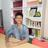#### RendiMdtm

Sep. 29, 2019
•#### Nisrinarozalini0511

Oct. 9, 2019
•#### ChiciWulandari

Nov. 1, 2019
•#### putrinatasya14

Nov. 6, 2019
•#### dilah_fara

Jan. 8, 2020
•#### hyakuyahartati

Apr. 28, 2020
•#### eritan

May. 21, 2020
•Jun. 15, 2020
•#### kautsar96

Jul. 1, 2020
•#### RosDiana31

Sep. 14, 2020
•#### ZulaikawidiaP

Oct. 22, 2020
•Dec. 7, 2020

Total views

95,987

On Slideshare

0

From embeds

0

Number of embeds

50,004

1,104

Shares

0